The modern language model with SOTA results on many NLP tasks is trained on large scale free text on the Internet. It is challenging to steer such a model to generate content with desired attributes. Although still not perfect, there are several approaches for controllable text generation, such as guided or learned decoding strategy, smart prompt design, or fine-tuning the model with various methods.

[Updated on 2021-02-01: Updated to version 2.0 with several work added and many typos fixed.]
[Updated on 2021-05-26: Add P-tuning and Prompt Tuning in the “prompt design” section.]
[Updated on 2021-09-19: Add “unlikelihood training”.]

There is a gigantic amount of free text on the Web, several magnitude more than labelled benchmark datasets. The state-of-the-art language models (LM) are trained with unsupervised Web data in large scale. When generating samples from LM by iteratively sampling the next token, we do not have much control over attributes of the output text, such as the topic, the style, the sentiment, etc. Many applications would demand a good control over the model output. For example, if we plan to use LM to generate reading materials for kids, we would like to guide the output stories to be safe, educational and easily understood by children.

How to steer a powerful unconditioned language model? In this post, we will delve into several approaches for controlled content generation with an unconditioned langage model. Note that model steerability is still an open research question. Each introduced method has certain pros & cons.

1. Apply guided decoding strategies and select desired outputs at test time.
2. Optimize for the most desired outcomes via good prompt design.
3. Fine-tune the base model or steerable layers to do conditioned content generation.

In the following discussion, we assume we have access to a pretrained generative language model $$p_\theta$$. The model has learned the distribution over token sequences by optimizing for the next token prediction: $$\mathcal{L}_\text{ML} = - \sum_t \log p_\theta(x_t \vert x_{<t})$$.

## Decoding Strategies

By adopting different decoding methods, we can place restrictions or preferences on the sampling process to alter the generated samples without modifying any model weights. Even though decoding strategies do not change the values of any trainable parameter, it is a quite important component.

### Common Decoding Methods

Since the final layer of the model predicts logits $$o$$ over the vocabulary space, the next token can be sampled by applying softmax with temperature $$T$$. The probability of sampling the $$i$$-th token is

$p_i \propto \frac{\exp(o_i / T)}{\sum_j \exp(o_j/T)}$

A low temperature would make the distribution sharper and a high value makes it softer.

Greedy search: Always pick the next token with the highest probability, equivalent to setting temperature $$T=0$$. However, it tends to create repetitions of phrases, even for well-trained models.

Beam search: It essentially does breadth-first search, one token per tree level, but with a limited bandwidth. At each level of the search tree, beam search keeps track of $$n$$ (named “beam width”) best candidates and expands all the successors of these candidates in the next level. Beam search could stop expanding a node if it hits the EOS (end-of-sentence) token.

However, maximization-based decoding does not guarantee high-quality generation.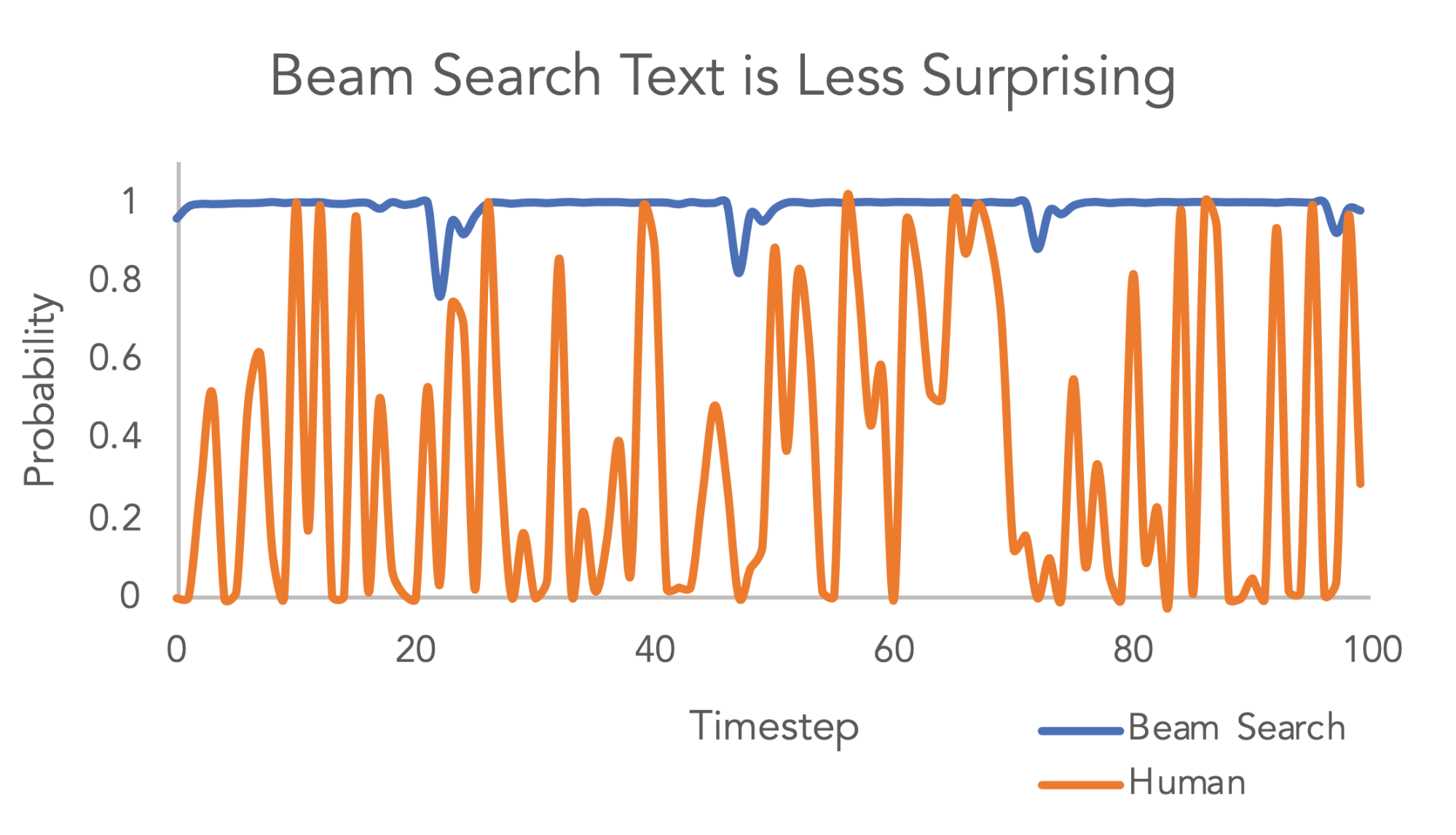Fig. 1. The probability assigned to the next token by beam search versus by humans. The human selected tokens have much higher variance in predicted probability and thus more surprising. (Image source: Holtzman et al. 2019)

Top-k sampling (Fan et al., 2018): At each sampling step, only the top $$k$$ most likely tokens are selected and the probability mass is redistributed among them. In Fan et al., 2018, the authors proposed to use top-k random sampling where the next token is randomly selected among the top $$k$$ most likely candidates and they argued that this approach can generate more novel and less repetitive content than beam search.

Nucleus sampling (Holtzman et al. 2019): Also known as “Top-p sampling”. One drawback of top-k sampling is that the predefined number $$k$$ does not take into consideration how skewed the probability distribution might be. The nucleus sampling selects the smallest set of top candidates with the cumulative probability exceeding a threshold (e.g. 0.95) and then the distribution is rescaled among selected candidates.

Both top-k and nucleus sampling have less repetitions with a proper set of hyperparameters.

Penalized sampling (Keskar et al. 2019): To avoid the common failure case of generating duplicate substrings, the CTRL paper proposed a new sampling method to penalize repetitions by discounting the scores of previously generated tokens. The probability distribution for the next token with repetition penalty is defined as:

$p_i = \frac{\exp(o_i / (T \cdot \mathbb{1}(i \in g)))}{\sum_j \exp(o_j / (T \cdot \mathbb{1}(j \in g)))} \quad \mathbb{1}(c) = \theta \text{ if the condition }c\text{ is True else }1$

where $$g$$ contains a set of previously generated tokens, $$\mathbb{1}(.)$$ is an identity function. $$\theta=1.2$$ is found to yield a good balance between less repetition and truthful generation.

### Guided Decoding

All the above standard decoding strategies sample tokens according to the predicted probability, with no additional information. Our preferences on topic or sentiment can be baked into the candidate ranking function to guide the sample generation by altering the candidate ranking score. The ranking score for token selection at each decoding step can be set as a combination of LM log-likelihood and a set of desired feature discriminators. The features are designed to quantify human preferences by heuristics (Ghazvininejad et al., 2017), supervised learning (Holtzman et al., 2018) or RL (Li et al., 2017).

Ghazvininejad et al. (2017) built a system called “Hafez” for generating poetry in desired style by adjusting sampling weights in beam search at decoding steps. The likelihood of sampling for the next token $$x_{t+1}$$ at step $$t$$ is augmented by a scoring function:

$\text{score}(x_{t+1}, b_t) = \text{score}(b_t) + \log p(x_{t+1}) + \color{green}{\sum_i \alpha_i f_i(x_{t+1})}$

where $$\log p(x_{t+1})$$ is the log-likelihood predicted by LM. $$\text{score}(b_t)$$ is the accumulated score of the already-generated words in the current beam state $$b_t$$. The green part can incorporate many different features for steering the style of the output. A set of feature functions $$f_i(.)$$ define the preferences and the associated weights $$alpha_i$$ work like “control knobs” that can be easily customized at decoding time. Features can measure a variety of attributes and can be easily combined; for example,

• whether $$x_{t+1}$$ exists in a bag of desired or banned topical words.
• whether $$x_{t+1}$$ indicates certain sentiments.
• whether $$x_{t+1}$$ is a repeated token (and thus $$f_i$$ needs to take the history as input too).
• the length of $$x_{t+1}$$ if longer or shorter words are in particular preferred.

Similar to Hafez, Baheti et al. (2018) manually designed features for ranking and altered the sampling distribution by appending similarity scores between topic distribution or embeddings of the context and the completion.

Holtzman et al. (2018) adopted a set of learned discriminators, each specializing in a different principle of communication guided by Grice’s maxims: quality, quantity, relation and manner. The discriminators learn to encode these desired principles by measuring repetition, entailment, relevance, and lexical diversity, respectively. Given some ground truth completion, all the discriminator models are trained to minimize the ranking log-likelihood, $$\log\sigma(f_i(y_g) - f_i(y))$$, because the gold continuation $$y_g$$ is expected to obtain a higher score than the generated one $$y$$. Here the weight coefficients $$\alpha_i$$ are also learned to minimize the score difference between the golden standard and the generated completion. Discriminative Adversarial Search (DAS; Scialom et al., 2020) is inspired by GAN and trains the discriminator to tell apart human created text from machine generated text. The discriminator predicts a label for each token instead of for the entire sequence. The discriminator logprob is added to the score to guide sampling towards the human-written style.

Meister et al. (2020) studied beam search in a regularized decoding framework:

$\mathbf{y}^* = \arg\max_{\mathbf{y}\in\mathcal{Y}} \big( \underbrace{\log p_\theta(\mathbf{y}\vert\mathbf{x})}_\text{MAP} - \underbrace{\lambda\mathcal{R}(\mathbf{y})}_\text{regularizer} \big)$

Since we expect maximum probability to have minimum surprise, the surprisal of a LM at time step $$t$$ can be defined as follows:

\begin{aligned} u_0(\texttt{BOS}) &= 0 \text{ ; BOS is a placeholder token for the beginning of a sentence.}\\ u_t(y) &= -\log P_\theta(y \vert \mathbf{x}, \mathbf{y}_{<t}) \text{ for }t \geq 1 \end{aligned}

The MAP (maximum a posteriori) part demands for sequences with maximum probability given context, while the regularizer introduces other constraints. It is possible a global optimal strategy may need to have a high-surprisal step occasionally so that it can shorten the output length or produce more low-surprisal steps afterwards.

Beam search has gone through the test of time in the field of NLP. The question is: If we want to model beam search as exact search in a regularized decoding framework, how should $$\mathcal{R}(\mathbf{y})$$ be modeled? The paper proposed a connection between beam search and the uniform information density (UID) hypothesis.

“The uniform information density hypothesis (UID; Levy and Jaeger, 2007) states that—subject to the constraints of the grammar—humans prefer sentences that distribute information (in the sense of information theory) equally across the linguistic signal, e.g., a sentence.”

In other words, it hypothesizes that humans prefer text with evenly distributed surprisal. Popular decoding methods like top-k sampling or nuclear sampling actually filter out high-surprisal options, thus implicitly encouraging the UID property in output sequences.

The paper experimented with several forms of regularizers:

1. Greedy: $$\mathcal{R}_\text{greedy}(\mathbf{y}) = \sum_{t=1}^{\vert\mathbf{y}\vert} \big(u_t(y_t) - \min_{y' \in \mathcal{V}} u_t(y') \big)^2$$; if set $$\lambda \to \infty$$, we have greedy search. Note that being greedy at each individual step does not guarantee global optimality.
2. Variance regularizer: $$\mathcal{R}_\text{var}(\mathbf{y}) = \frac{1}{\vert\mathbf{y}\vert}\sum_{t=1}^{\vert\mathbf{y}\vert} \big(u_t(y_t) - \bar{u} \big)^2$$ , where $$\bar{u}$$ is the average surprisal over all timesteps. It directly encodes the UID hypothesis.
3. Local consistency: $$\mathcal{R}_\text{local}(\mathbf{y}) = \frac{1}{\vert\mathbf{y}\vert}\sum_{t=1}^{\vert\mathbf{y}\vert} \big(u_t(y_t) - u_{t-1}(y_{t-1}) \big)^2$$; this decoding regularizer encourages adjacent tokens to have similar surprisal.
4. Max regularizer: $$\mathcal{R}_\text{max}(\mathbf{y}) = \max_t u_t(y_t)$$ penalizes the maximum compensation of surprisal.
5. Squared regularizer: $$\mathcal{R}_\text{square}(\mathbf{y}) = \sum_{t=1}^{\vert\mathbf{y}\vert} u_t(y_t)^2$$ encourages all the tokens to have surprisal close to 0.

An experiment with greedy regularizers showed that larger $$\lambda$$ results in better performance (e.g. measured by BLEU for NMT task) and lower std dev of surprisal.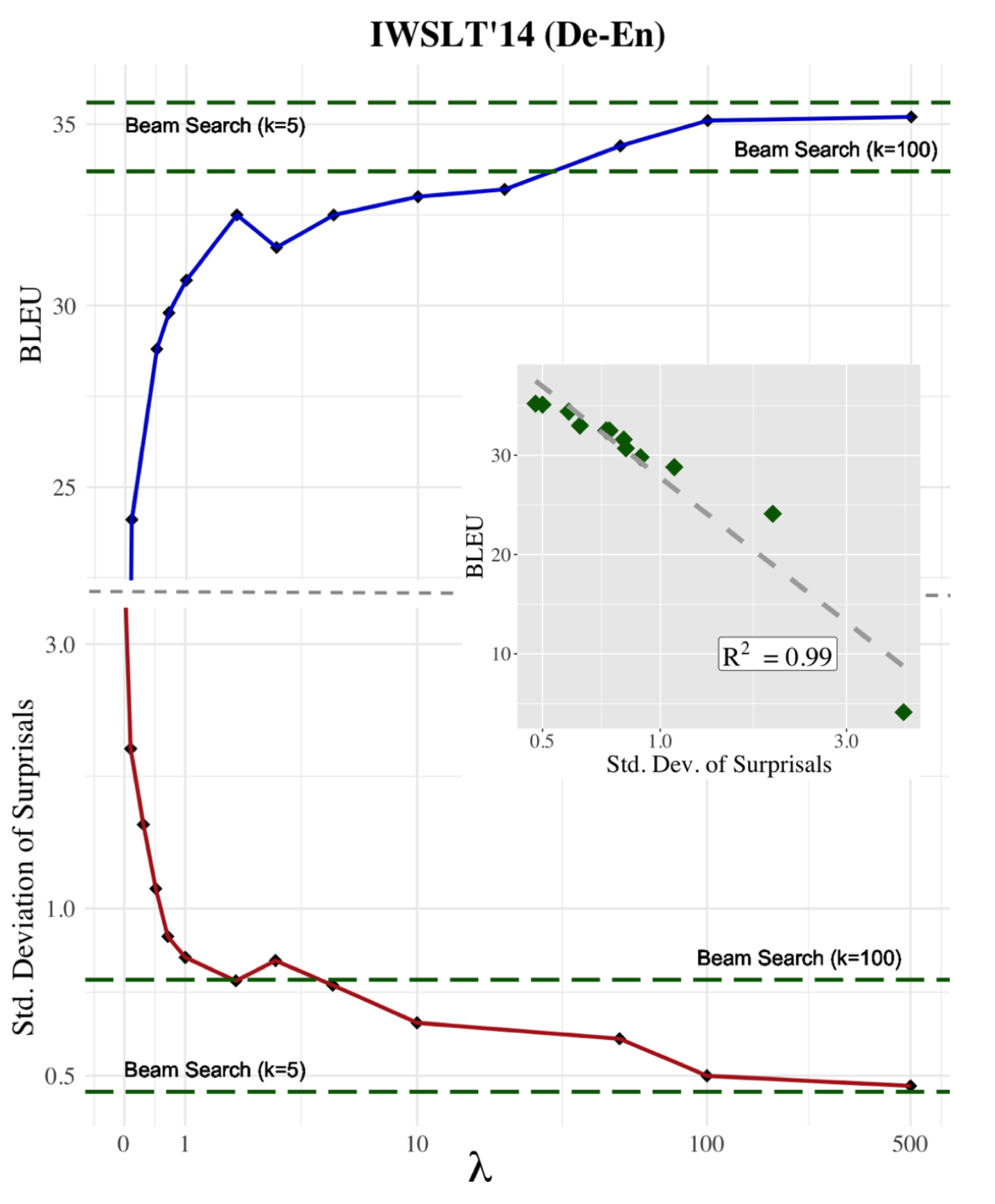Fig. 2. The plot of BLEU and std. dev of surprisals as functions of the strength of the regularizer $$\lambda$$. The subgraph in grey shows the relationship between BLEU and surprisal std. dev. (Image source: Meister et al. 2020)

A default beam search would have text generation of decreased quality when beam size increases. Regularized beam search greatly helps alleviate this issue. A combined regularizer further improves the performance. In their experiments for NMT, they found $$\lambda=5$$ for greedy and $$\lambda=2$$ for squared work out as the optimal combined regularizer.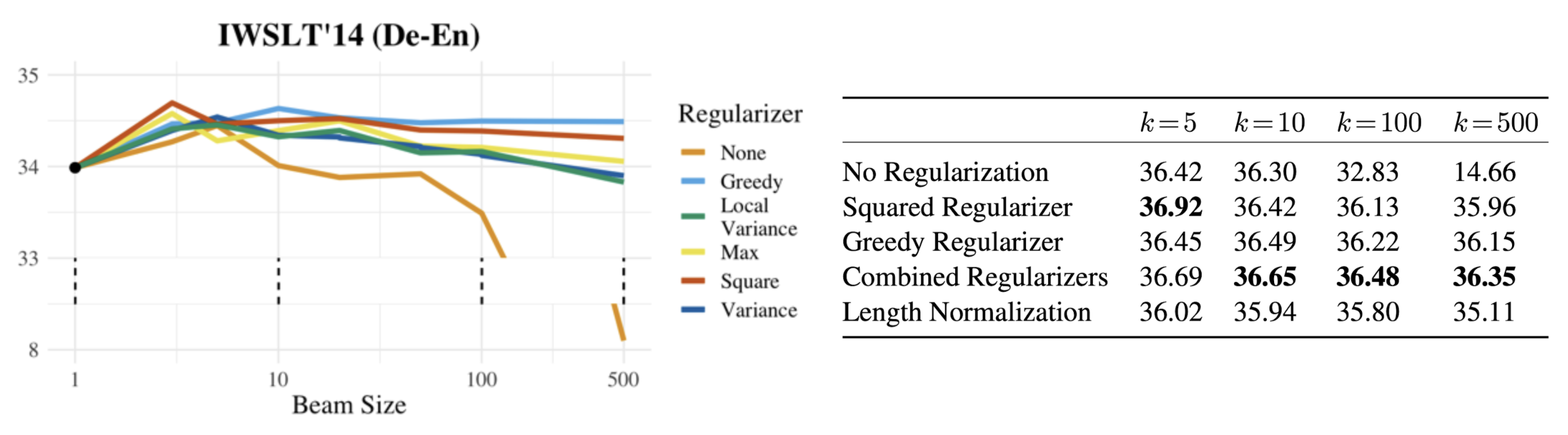Fig. 3. The plot of BLEU of a function of beam size (left) and BLEU scores for translations created by different regularized decoding strategies. (Image source: Meister et al. 2020)

Guided decoding essentially runs a more expensive beam search where the sampling probability distribution is altered by side information about human preferences.

### Trainable Decoding

Given a trained language model, Gu et al (2017) proposed a trainable greedy decoding algorithm to maximize an arbitrary objective for sampling sequences. The idea is based on the noisy, parallel approximate decoding (NPAD). NPAD injects unstructured noise into the model hidden states and runs noisy decoding multiple times in parallel to avoid potential degradation. To take a step further, trainable greedy decoding replaces the unstructured noise with a learnable random variable, predicted by a RL agent that takes the previous hidden state, the previous decoded token and the context as input. In other words, the decoding algorithm learns a RL actor to manipulate the model hidden states for better outcomes.

Grover et al. (2019) trained a binary classifier to distinguish samples from data distribution and samples from the generative model. This classifier is used to estimate importance weights for constructing a new unnormalized distribution. The proposed strategy is called likelihood-free importance weighting (LFIW).

Let $$p$$ be the real data distribution and $$p_\theta$$ be a learned generative model. A classical approach for evaluating the expectation of a given function $$f$$ under $$p$$ using samples from $$p_\theta$$ is to use importance sampling.

$\mathbb{E}_{\mathbf{x}\sim p} [f(\mathbf{x})] = \mathbb{E}_{\mathbf{x}\sim p_\theta} \Big[\frac{p(\mathbf{x})}{p_\theta(\mathbf{x})} f(\mathbf{x})\Big] \approx \frac{1}{N} \sum_{i=1}^N w(\mathbf{x}_i)f(\mathbf{x}_i)$

However, $$p(\mathbf{x})$$ can only be estimated via finite datasets. Let $$c_\phi: \mathcal{X} \to [0,1]$$ be a probabilistic binary classifier for predicting whether a sample $$\mathbf{x}$$ is from the true data distribution ($$y=1$$). The joint distribution over $$\mathcal{X}\times\mathcal{Y}$$ is denoted as $$q(\mathbf{x}, y)$$.

$q(\mathbf{x}\vert y) = \begin{cases} p_\theta(\mathbf{x}) & \text{ if }y=0\text{; predicted to be generated data} \\ p(\mathbf{x}) & \text{ otherwise; from the true data distribution} \end{cases}$

Then if $$c_\phi$$ is Bayes optimal, the importance weight can be estimated by:

$w_\phi(\mathbf{x}) = \frac{p(\mathbf{x})}{p_\theta(\mathbf{x})} = \frac{q(\mathbf{x} \vert y=1)}{q(\mathbf{x} \vert y=0)} = \frac{q(y=0)}{q(y=1)} \frac{q(y=1 \vert \mathbf{x})}{q(y=0 \vert \mathbf{x})} = \gamma \frac{c_\phi(\mathbf{x})}{1 - c_\phi(\mathbf{x})}$

where $$\gamma = \frac{q(y=0)}{q(y=1)} > 0$$ is a fixed odd ratio.

Since we cannot learn a perfect optimal classifier, the importance weight would be an estimation $$\hat{w}_\phi$$. A couple of practical tricks can be applied to offset cases when the classifier exploits artifacts in the generated samples to make very confident predictions (i.e. very small importance weights):

1. Self-normalization: normalize the weight by the sum $$\hat{w}_\phi(\mathbf{x}_i) / \sum_{j=1}^N \hat{w}_\phi(\mathbf{x}_j)$$.
2. Flattening: add a power scaling parameter $$\alpha > 0$$, $$\hat{w}_\phi(\mathbf{x}_i)^\alpha$$.
3. Clipping: specify a lower bound $$\max(\hat{w}_\phi(\mathbf{x}_i), \beta)$$.

To sample from an importance resampled generative model, $$\mathbf{x}\sim p_{\theta, \phi}(\mathbf{x}) \propto p_\theta(\mathbf{x})\hat{w}_\phi(\mathbf{x})$$, they adopt SIR (Sampling-Importance-Resampling),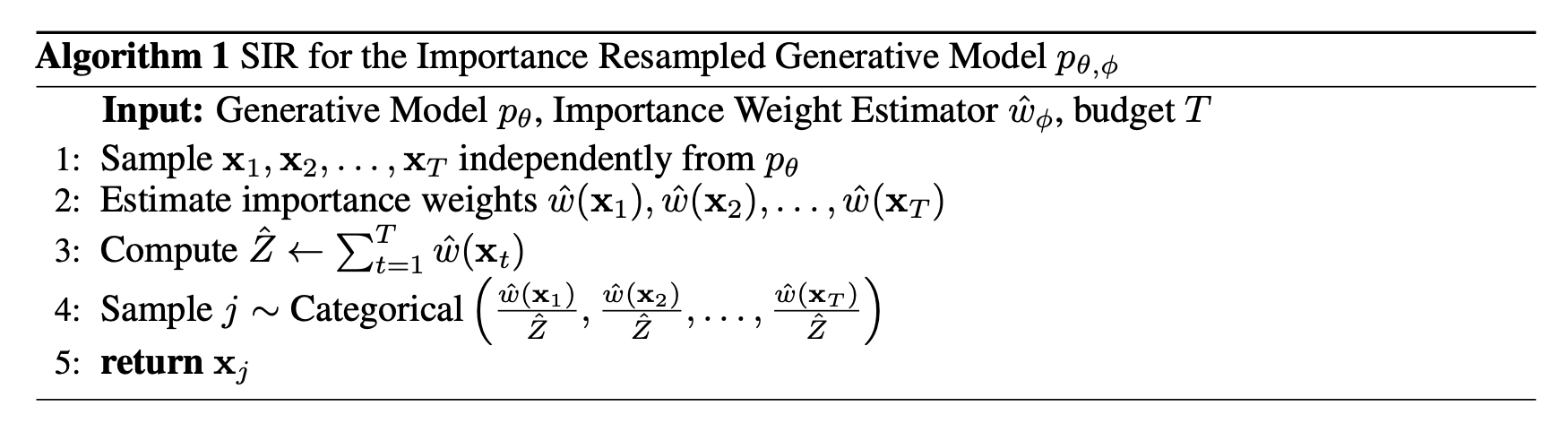Fig. 4. The algorithm for sampling from a generative model according to importance weights $$\hat{w}(\mathbf{x}_i)$$ using SIR. (Image source: Grover et al., 2019))

Deng et al., 2020 proposed to learn a EBM to steer a LM in the residual space, $$P_\theta(x) \propto P_\text{LM}(x)\exp(-E_\theta(x))$$, where $$P_\theta$$ is the joint model; $$E_\theta$$ is the residual energy function to be learned. If we know the partition function $$Z$$, we can model the generative model for generative a sequence $$x_{p+1}, \dots, x_T$$ as:

$P_\theta(x_{p+1:T}\vert x_{1:p}) = \frac{P_\text{LM}(x_{p+1:T}\vert x_{1:p}) \exp(-E_\theta(x_{1:T}))}{Z_\theta(x_{1:p})}$

The goal is to learn the parameters of the energy function $$E_\theta$$ such that the joint model $$P_\theta$$ gets closer to the desired data distribution. The residual energy function is trained by noise contrastive estimation (NCE), considering $$P_\theta$$ as the model distribution and $$P_\text{LM}$$ as the noise distribution:

$\theta = \arg\max_{\theta} \mathbb{E}_{x^+ \sim P_\text{data}} \log\frac{1}{1+\exp(E_\theta(x^+))} + \mathbb{E}_{x^- \sim P_\text{LM}} \log\frac{1}{1+\exp(-E_\theta(x^-))}$

However, the partition function is intractable in practice. The paper proposed a simple way to first sample from the original LM and then to resample from them according to the energy function. This is unfortunately quite expensive.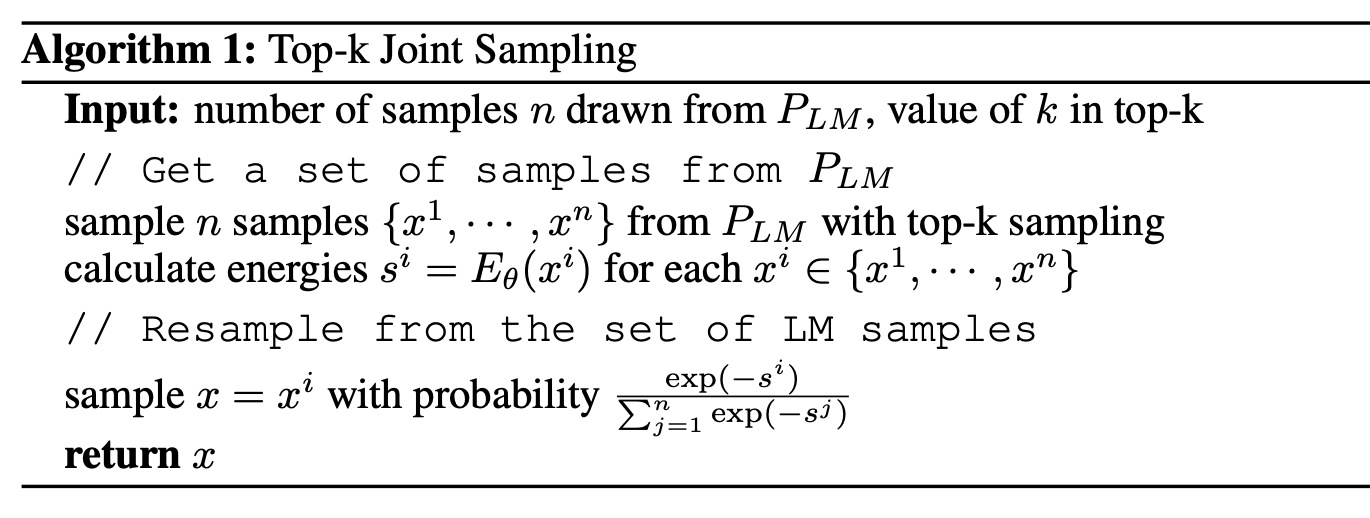Fig. 5. Top k samples from the base LM are resampled according to the residual energy function. (Image source: Deng et al., 2020)

## Smart Prompt Design

Large language models have been shown to be very powerful on many NLP tasks, even with only prompting and no task-specific fine-tuning (GPT2, GPT3). The prompt design has a big impact on the performance on downstream tasks and often requires time-consuming manual crafting. For example, factual questions can gain a big boost with smart prompt design in “closed-book exam” (Shin et al., 2020, Jiang et al., 2020)). I’m expecting to see an increasing amount of literature on automatic smart prompt design.

AutoPrompt (Shin et al., 2020; code) is a method to automatically create prompts for various tasks via gradient-based search. AutoPrompt constructs a prompt by combining the original task inputs $$x$$ with a collection of trigger tokens $$x_\text{trig}$$ according to a template $$\lambda$$. The trigger tokens are shared across all inputs and thus universally effective.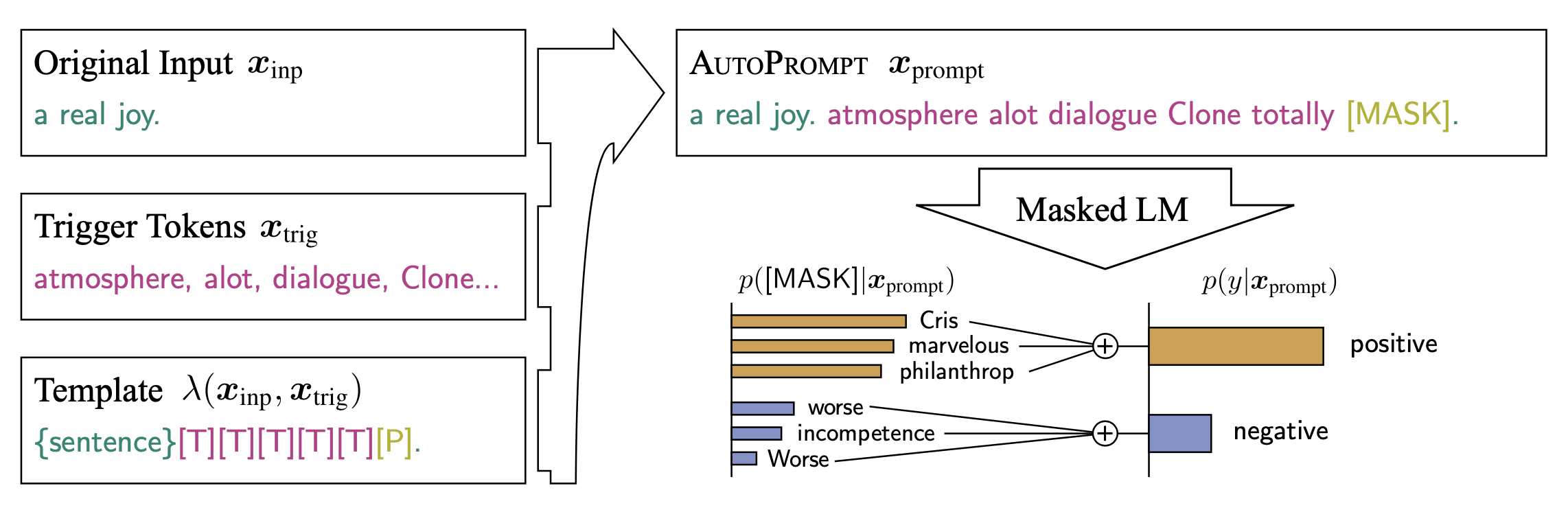Fig. 6. The overview of AutoPrompt. The trigger tokens are retrieved to optimize for the target outputs across all inputs. (Image source: Shin et al., 2020)

The universal trigger tokens are identified using a gradient-guided search strategy same as in Wallace et al., 2019. The universal setting means that the trigger tokens $$x_\text{trig}$$ can optimize for the target output $$\tilde{y}$$ for all inputs from a dataset:

$x_\text{trig} = \arg\min_{x’_\text{trig}} \mathbb{E}_{x\sim\mathcal{X}} [\mathcal{L}(\tilde{y}, f(x’_\text{trig}; x))]$

The search operates in the embedding space. The embedding of every trigger token $$e_{\text{trig}_i}$$ is first initialized to some default value and then gets updated to minimize the first-order Taylor expansion of the task-specific loss around the current token embedding:

$e^{(t+1)}_\text{trig} = \arg\min_{e\in\mathcal{V}} [e - e^{(t)}_{\text{trig}_i}]^\top \nabla_{e^{(t)}_{\text{trig}_i}} \mathcal{L}$

where $$\mathcal{V}$$ refers to the embedding matrix of all the tokens. $$\nabla_{e^{(t)}_{\text{trig}_i}} \mathcal{L}$$ is the average gradient of the task loss over a batch at iteration $$t$$. We can brute-force the optimal $$e$$ by a $$\vert \mathcal{V} \vert d$$-dimensional dot product, which is cheap and can be computed in parallel.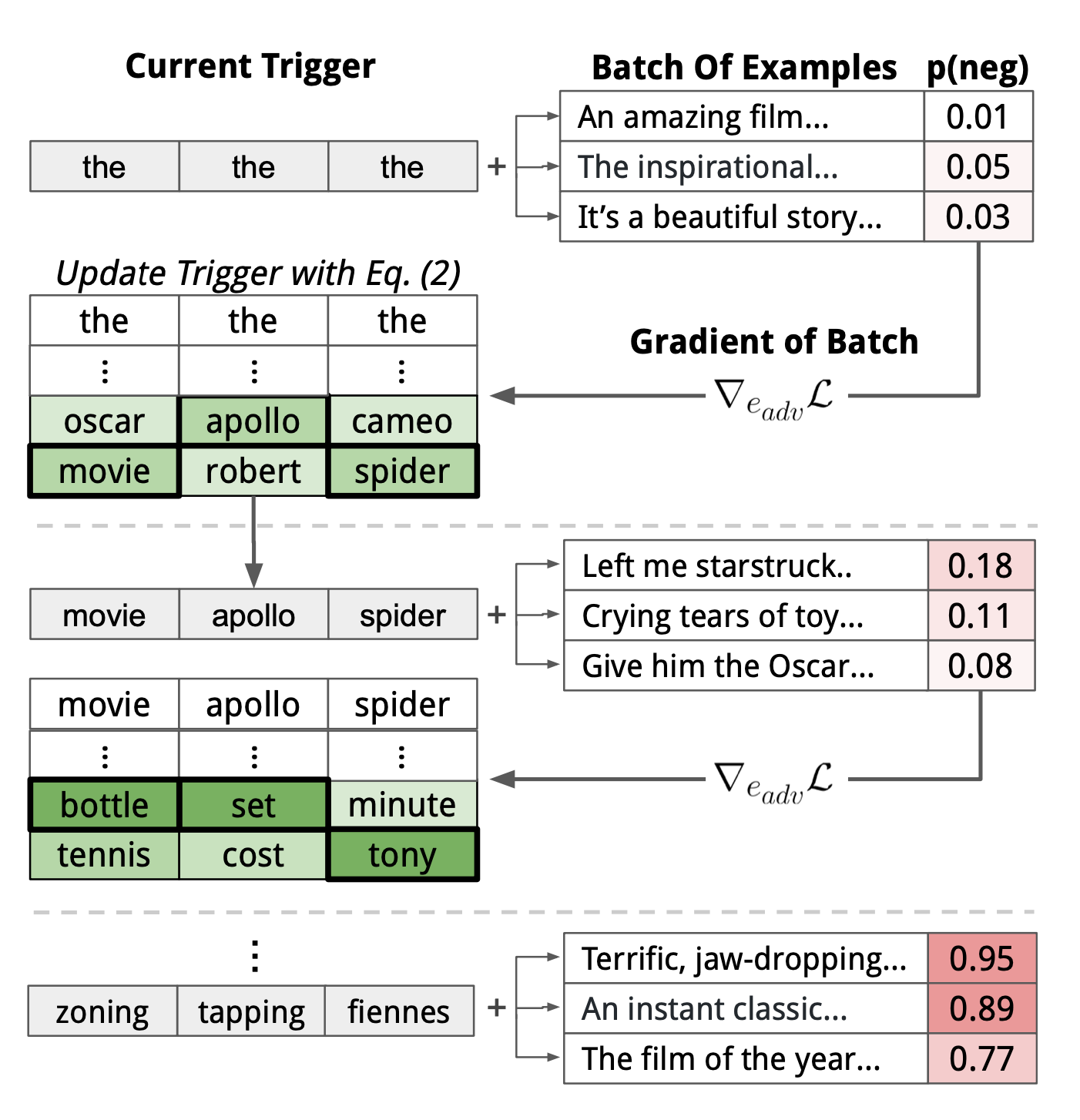Fig. 7. We search for trigger tokens by updating their embeddings with the gradient of the task loss per batch. (Image source: Wallace et al., 2019)

The above token replacement method can be augmented with beam search. When looking for the optimal token embedding $$e$$, we can pick top-$$k$$ candidates instead of a single one, searching from left to right and score each beam by $$\mathcal{L}$$ on the current data batch.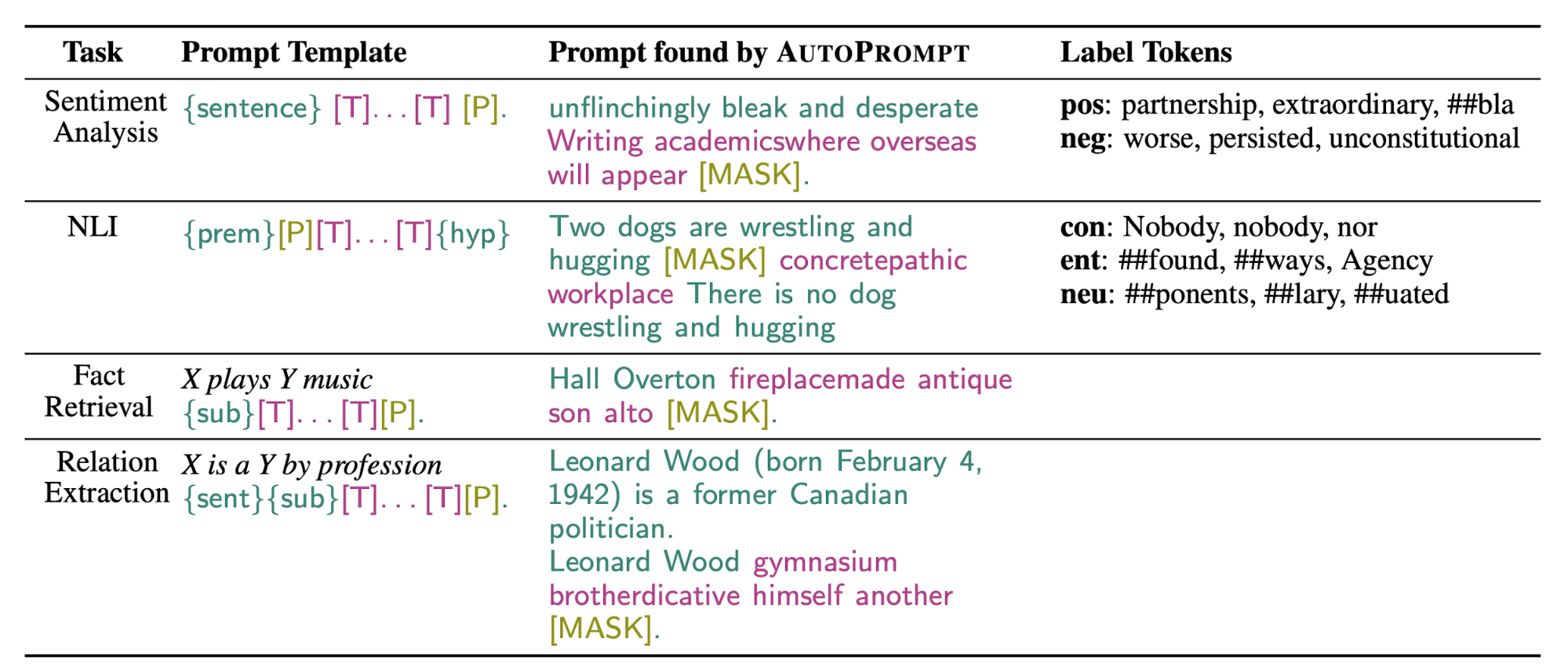Fig. 8. Example prompts discovered by AutoPrompt for different tasks. (Image source: Shin et al., 2020)

Smart prompt design essentially produces efficient context that can lead to desired completion. Motivated by this observation, Li & Liang (2021) proposed Prefix-Tuning which assigns a small number of trainable parameters at the beginning of an input sequence (named “prefix”) to steer a LM, $$[\text{PREFIX}; x; y]$$. Let $$\mathcal{P}_\text{idx}$$ be a set of prefix indices and $$\text{dim}(h_i)$$ be the embedding size. The prefix parameters $$P_\theta$$ has the dimension $$\vert\mathcal{P}_\text{idx}\vert \times \text{dim}(h_i)$$ and the hidden state takes the form:

$h_i = \begin{cases} P_\theta[i,:], & \text{if }i \in \mathcal{P}_\text{idx}\\ \text{LM}_\phi(z_i, h_{<i}), & \text{otherwise} \end{cases}$

Note that only $$P_\theta$$ is trainable and the LM parameters $$\phi$$ is frozen during training.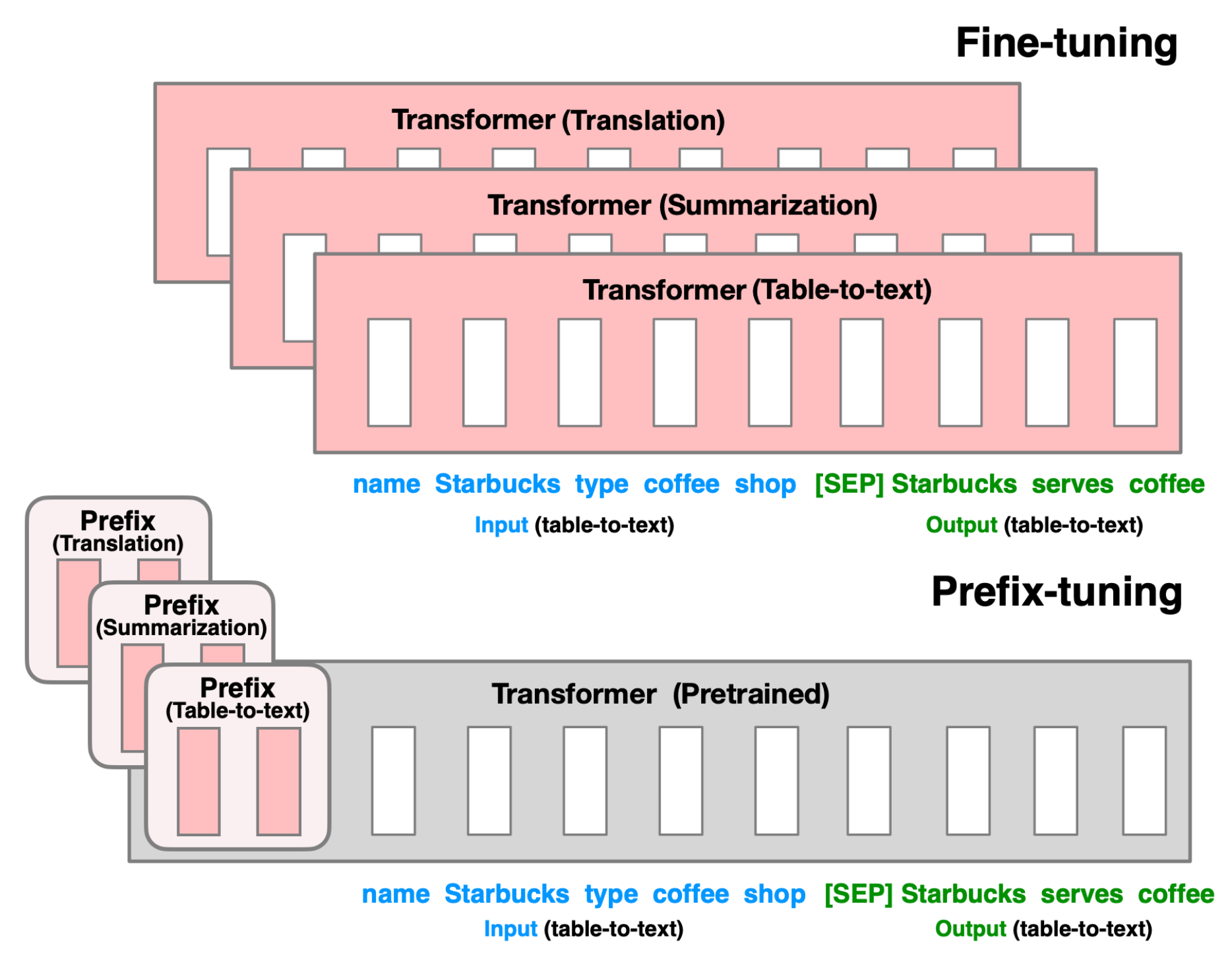Fig. 9. Illustrations of fine-tuning versus prefix-tuning. (Image source: Li & Liang 2021)

The prefix parameters do not tie to any embeddings associated with the real words and thus they are more expressive for steering the context. Direct optimizing $$P_\theta$$ unfortunately results in poor performance. To reduce the difficulty associated with high dimensionality training, the matrix $$P_\theta$$ is reparameterized by a smaller matrix $$P'_\theta \in \mathbb{R}^{\vert\mathcal{P}_\text{idx}\vert \times c}$$ and a large feed forward network $$\text{MLP}_\theta \in \mathbb{R}^{c\times \text{dim}(h_i)}$$.

The performance increases with the prefix length $$\vert\mathcal{P}_\text{idx}\vert$$ up to some value. And this value varies with tasks.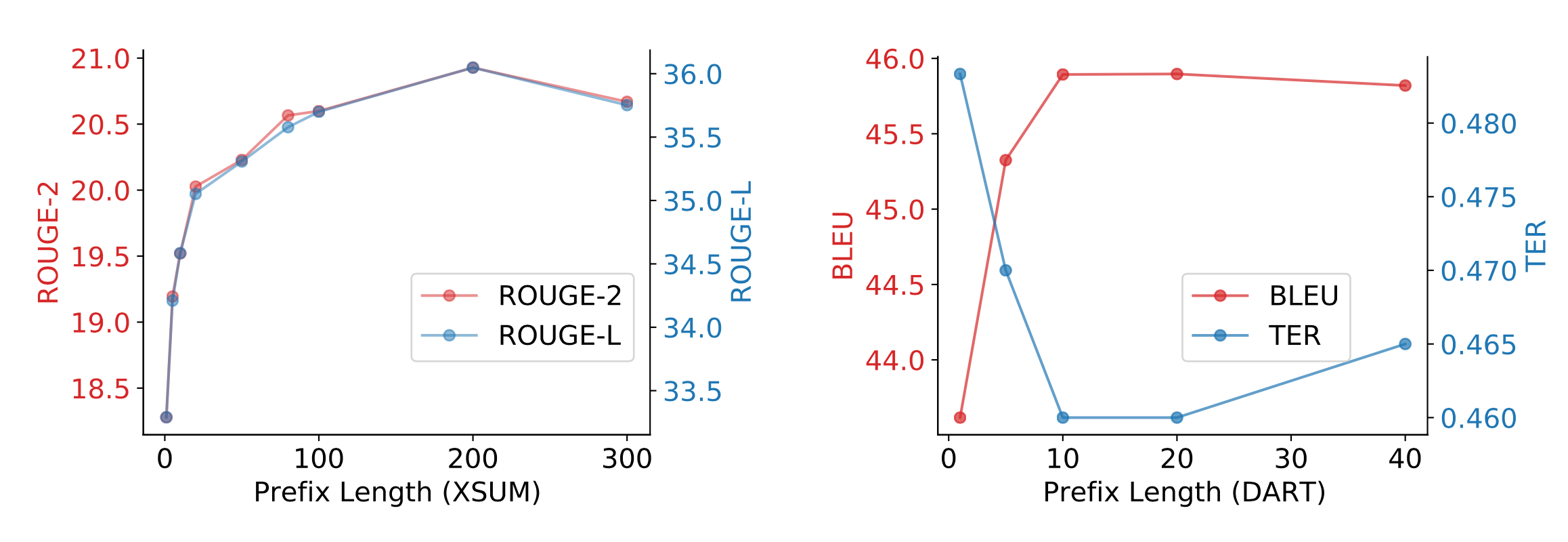Fig. 10. Task performance, summarization (left) and table-to-text (right), as a function of prefix length. (Image source: Li & Liang 2021)

A few other interesting learnings from their ablation studies include:

• Tuning only the embedding layer (without prefix) is not sufficiently expressive.
• Placing the trainable parameter between $$x$$ and $$y$$, $$[x; \text{INFIX}; y]$$, slightly underperforms prefix-tuning, likely because it only affects the context for $$y$$ while prefix affects both.
• Random initialization of $$P_\theta$$ leads to low performance with high variance. In contrast, initializing $$P_\theta$$ with activations of real words improves generation, even the words are irrelevant to the task.

Fine-tuned models achieve better task performance but they can fail in the low data regime. Both AutoPrompt and Prefix-Tuning were found to outperform fine-tuning in the regime where the training dataset is small (i.e. $$10^2-10^3$$ samples). As an alternative to fine-tuning, prompt design or learning the context embedding is much cheaper. AutoPrompt improves the accuracy for sentiment classification a lot more than manual prompts and achieves similar performance as linear probing. For the NLI task, AutoPrompt obtains higher accuracy than linear probing. It is able to retrieve facts more accurately than manual prompts too. In low data regime, Prefix-Tuning achieves performance comparable with fine-tuning on table-to-text generation and summarization.

Two successive works, P-tuning (Liu et al. 2021; code) and Prompt Tuning (Lester et al. 2021), follow the similar idea of explicit training continuous prompt embeddings but with a few different choices over the trainable parameters and architecture. Different from Prefix-Tuning which concatenates continuous prompt tokens in every hidden state layer of the transformer, both P-tuning and Prompt Tuning non-invasively add continuous prompts only in the input to work well.

Let $$[P_i]$$ be the $$i$$-th token in the prompt template of P-tuning (Liu et al. 2021), we can denote a prompt as a sequence $$T=\{[P_{0:i}], \mathbf{x}, [P_{i+1:m}], \mathbf{y}\}$$. Each token $$[P_i]$$ does not have to be a real token in the model vocabulary (“pseudo-token”), and thus the encoded template $$T^e$$ looks like the following and the pseudo-token hidden state can be optimized with gradient descent.

$T^e = \{ h_0, \dots, h_i, \text{embed}(\mathbf{x}), h_{i+1}, \dots, h_m, \text{embed}(\mathbf{y})\}$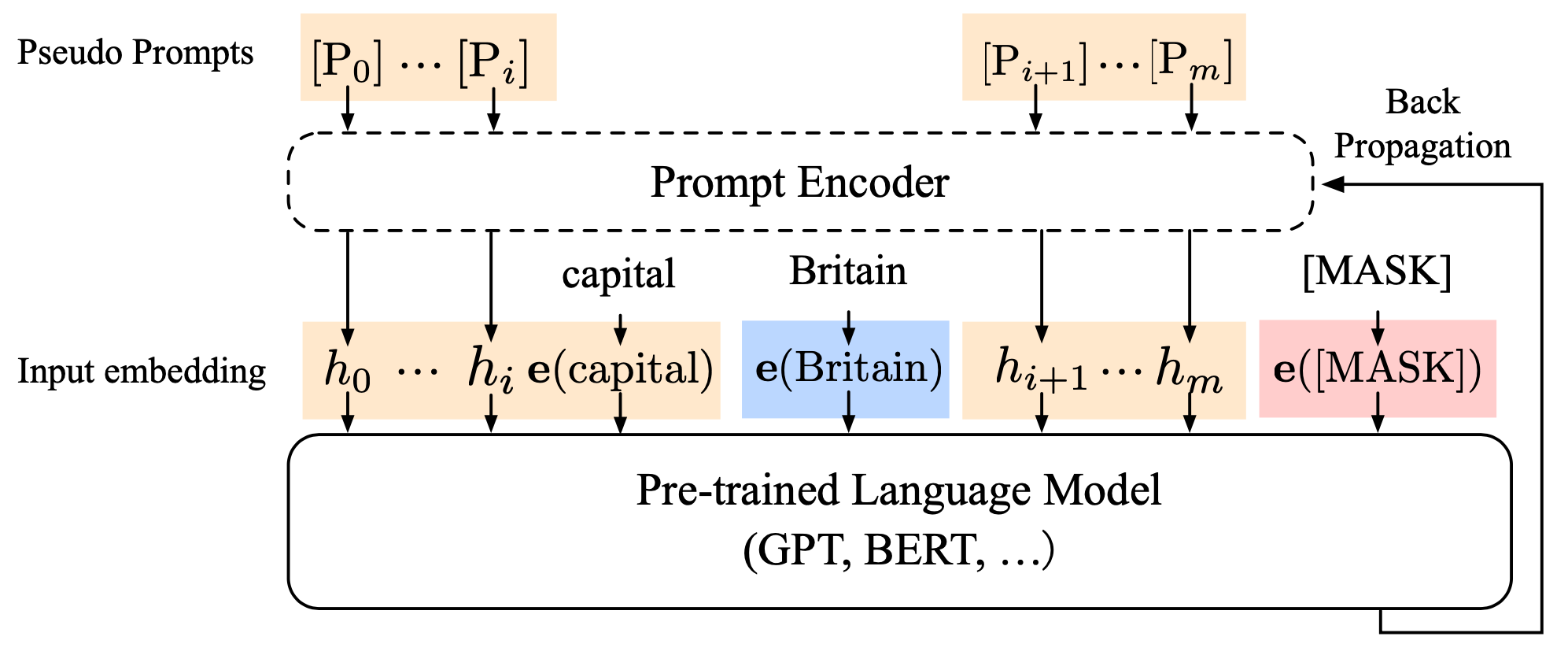Fig. 11. The illustration of P-tuning. Sometimes, adding a few task-related anchor tokens, such as “capital” in the figure, can bring further improvement. (Image source: Liu et al. 2021)

There are two major optimization challenges in P-tuning:

1. Discreteness: The word embedding of a pretrained language model are highly discrete. It is hard to optimize $$h_i$$ if they are intialized at random.
2. Association: $$h_i$$ should be dependent on each other. Thus they develop a mechanism to model this dependency by training a light-weighted LSTM-based prompt encoder:
$h_i = \text{MLP}([\text{LSTM}(h_{0:i}): \text{LSTM}(h_{i:m})])$

P-tuning is more flexible than prefix-tuning, as it inserts trainable tokens in the middle of a prompt not just at the beginning. The usage of task-specific anchor tokens is like combining manual prompt engineering with trainable prompts.

Prompt Tuning (Lester et al. 2021) largely simplifies the idea of prefix tuning by only allowing an additional $$k$$ tunable tokens per downstream task to be prepended to the input text. The conditional generation is $$p_{\theta, \theta_P}(Y \vert [P; X])$$, where $$P$$ is the “pseudo prompt” with parameters $$\theta_P$$ trainable via back-propagation. Both $$X$$ and $$P$$ are embedding vectors and we have $$X \in \mathbb{R}^{n \times d^e}, P \in \mathbb{R}^{k \times d^e}$$ and $$[P;X] \in \mathbb{R}^{(n+k) \times d^e}$$, where $$d^e$$ is the embedding space dimensionality.

• Prompt tuning produces competitive results as model fine-tuning when the model gets large (billions of parameters and up). This result is especially interesting given that large models are expensive to fine-tune and execute at inference time.
• With learned task-specific parameters, prompt tuning achieves better transfer learning when adapting to new domains. It outperforms fine-tuning on domain shift problems.
• They also showed that prompt ensembling of multiple prompts for the same task introduces further improvement.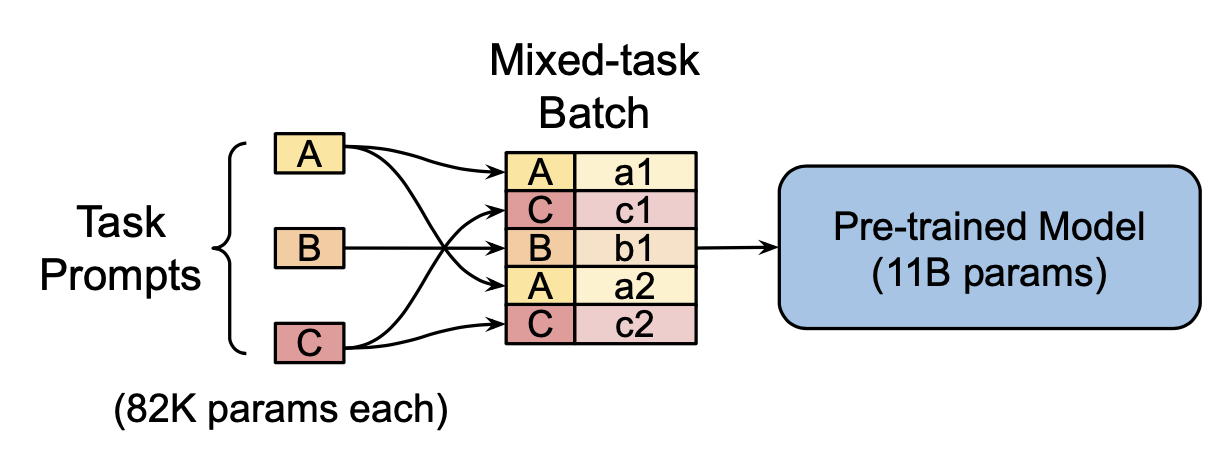Fig. 12. The illustration of how Prompt Tuning works. (Image source: Lester et al. 2021)

The experiments investigated several prompt initialization schemes:

1. Random initialization by uniformly sampling from [-0.5, 0.5];
2. Sample embeddings of top 5000 common tokens;
3. Use the embedding values of the class label strings. If we don’t have enough class labels to initialize the soft-prompt, we fall back to scheme 2. Random initialization performs noticeably worse than the other two options.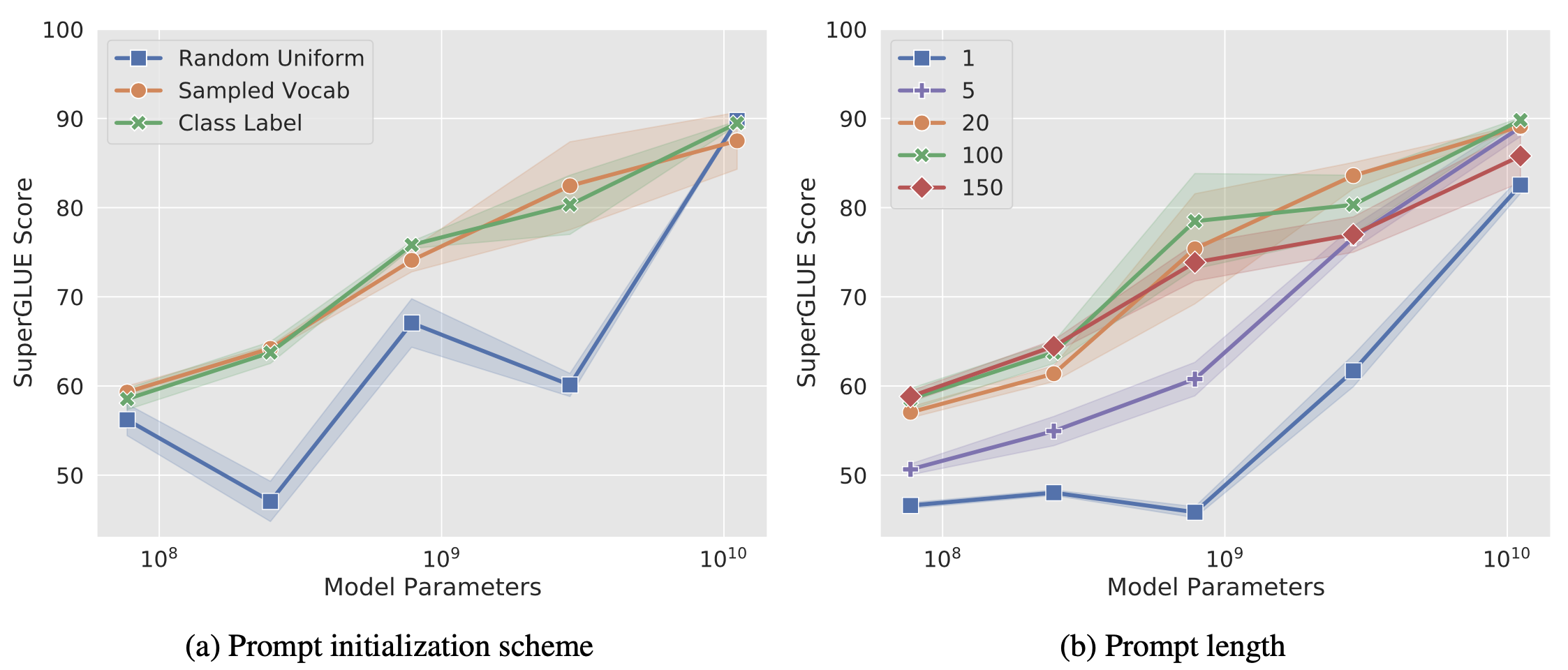Fig. 13. The effect of (a) different prompt initialization schemes and (b) different prompt lengths. (Image source: Lester et al. 2021)

The pre-training objectives also have a big impact on the quality of prompt tuning. T5’s “span corruption” is not a good option here.

Prompt tuning is found to be less likely to overfit to a specific dataset. To evaluate the robustness to data shifting problem, they trained the model on one dataset of one task and evaluated it on the test dataset but in a different domain. Prompt tuning is more resilient and can generalize to different domains better.Fig. 14. Prompt tuning is more resilient to domain shift between train and test sets. (Image source: Lester et al. 2021)

Paraphrasing is a quick way to explore more prompts similar to the known version, which can be done via back-translation. Using back-translation, the initial prompt is translated into $$B$$ candidates in another language and then each is translated back into $$B$$ candidates in the original language. The resulting total $$B^2$$ candidates are scored and ranked by their round-trip probabilities.

Ribeiro et al (2018) identified semantically equivalent adversaries (SEA) by generating a variety of paraphrases $$\{x'\}$$ of input $$x$$ until it triggers a different prediction of target function $$f$$:

\begin{aligned} SEA(x, x') &= \mathbb{1}[\text{SemEq}(x, x') \land f(x) \neq f(x')] \\ \text{where SemEq}(x, x') &= \mathbb{1}[\min\Big(1, \frac{p(x'\vert x)}{p(x\vert x)} \Big) \geq \tau] \end{aligned}

The rules extracted from SEA are considered as “bugs” in the model. Applying those rules as data augmentation in model training helps robustify the model and fix bugs.

Jiang et al (2020) attempts to validate whether a trained language model knows certain knowledge by automatically discovering better prompts to query. Within the scope of knowledge retrieval where factual knowledge is represented in the form of a triple $$\langle x, r, y \rangle$$ (subject, relation, object). The prompts can be mined from training sentences (e.g. Wikipedia description) or expanded by paraphrase.

Interestingly some small modifications in the prompts may lead to big gain, as shown in Fig. X.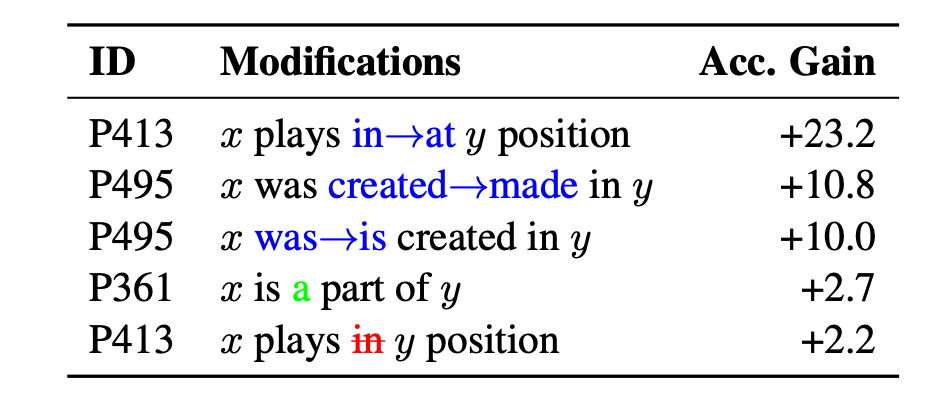Fig. 15. Small modifications in prompt templates can lead to big performance gains: replacement in blue, insertion in green, deletion in red. (Image source: Jiang et al., 2020)

## Fine-tuning

Fine-tuning is an intuitive way to guide a LM to output desired content, commonly by training on supervised datasets or by RL. We can fine-tune all the weights in the model or restrict the fine-tuning to only top or additional layers.

### Conditional Training

Conditional training aims to learn a generative model conditioned on a control variable $$z$$, $$p(y \vert x, z)$$.

Fan et al (2018) trained a conditional language model for 2-step story generation. First, a model outputs the story sketch and then a story writing model creates a story following that sketch. The mechanism of conditioning on the sketch is implemented by a fusion model architecture. The fusion model enforces a form of residual learning that allows the story writing model to focus on learning what the first sketch generation model is missing. Also for story generation, Peng et al (2018) experimented with an ending valence-conditioned story generator LM, $$p(x_t \vert x_{<t}, z)$$ where $$z$$ is the label of the story ending (sad, happy or neutral). Their language model is a bidirectional LSTM and the label is mapped into a learned embedding which then blends into the LSTM cell.

CTRL (Keskar et al., 2019; code) aims to train a language model conditioned control code $$z$$ using controllable datasets. CTRL learns the conditioned distribution $$p(x \vert z)$$ by training on raw text sequences with control code prefixes, such as [horror], [legal], etc. Then the learned model is able to generate text with respect to the prompt prefix. The training data contains Wikipedia, OpenWebText, books, Amazon reviews, reddit corpus and many more, where each dataset is assigned with a control code and subreddit in the reddit corpus has its own topic as control code.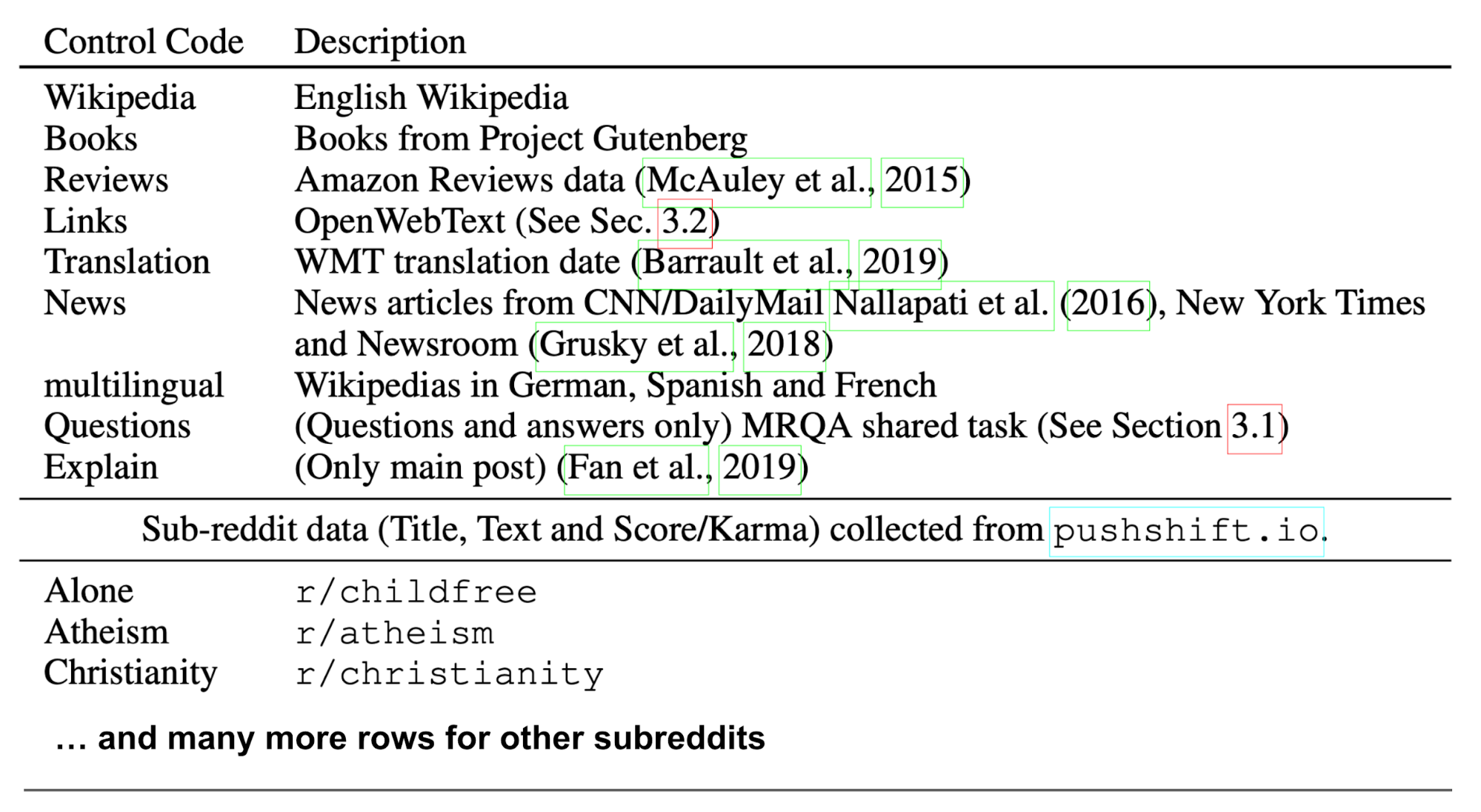Fig. 16. Datasets used for training CTRL and associated control codes. (Image source: Edited from Table 7 in Keskar et al., 2019)

The control code also can be used for domain annotation given tokens, because $$p(z \vert x) \propto p(x \vert z) p(z)$$, assuming the prior over domains is uniform. One limitation of CTRL is the lack of control for what not to generate (e.g. avoid toxicity).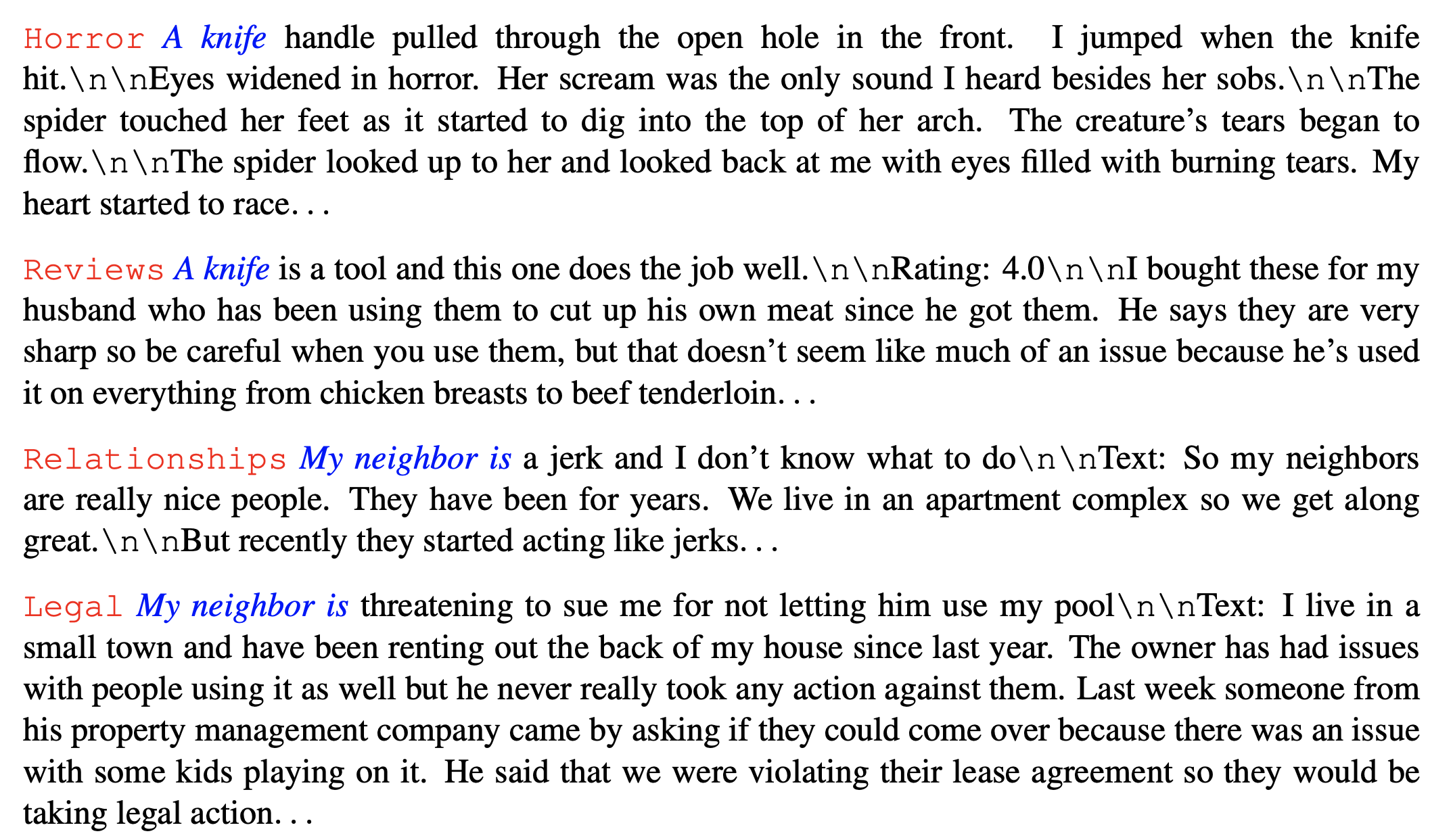Fig. 17. The examples of conditioned sample generation by CTRL. (Image source: Keskar et al., 2019)

Note that CTRL trains a transformer model from scratch. However, labelling all the text within the same dataset with the same control code (e.g. All the wikipedia articles have “wikipedia” as control code) feels quite constrained. Considering that often we need highly customized control codes but only have a limited amount of labelled data, I would expect fine-tuning an unconditional LM with a small labelled dataset in the same way as CTRL to work out well too. Although how much data is needed and how good the sample quality might be are subject to experimentation.

### RL Fine-tuning

Fine-tuning a sequential model with RL regarding any arbitrary and possibly non-differentiable reward function has been proved to work well years ago (Ranzato et al., 2015). RL fine-tuning can resolve several problems with teacher forcing method. With teacher forcing, the model only minimizes a maximum-likelihood loss at each individual decoding step during training but it is asked to predict the entire sequence from scratch at test time. Such a discrepancy between train and test could lead to exposure bias and accumulated error. In contrast, RL fine-tuning is able to directly optimize task-specific metrics on the sequence level, such as BLEU for translation (Ranzato et al., 2015, Wu et al., 2016, Nguyen et al., 2017), ROUGE for summarization (Ranzato et al., 2015, Paulus et al., 2017, Wu and Hu, 2018) and customized metric for story generation (Tambwekar et al., 2018).

Ranzato et al (2015) applied REINFORCE to train RNN models for sequence generation tasks. The model is first trained to predict the next token using cross-entropy loss (ML loss) and then fine-tuned alternatively by both ML loss and REINFORCE (RL loss). At the second fine-tuning stage, the number of training steps for next-token prediction is gradually decreasing until none and eventually only RL loss is used. This sequence-level RL fine-tuning was shown by experiments to lead to great improvements over several supervised learning baselines back then.

Google implemented the similar approach in their neural machine translation system (Wu et al., 2016) and Paulus et al (2017) adopted such approach for summarization task. The training objective contains two parts, ML loss for next token prediction, $$\mathcal{L}_\text{ML} = \sum_{(x, y^*)\sim\mathcal{D}} \log p_\theta(y^* \vert x)$$, and RL loss $$\mathcal{L}_\text{RL}$$ for maximizing the expected reward where the reward per sequence is measured by BLEU or ROUGE. The model is first trained with $$\mathcal{L}_\text{ML}$$ until convergence and then fine-tuned with a linear combination of two losses, $$\mathcal{L}_\text{mix} = \alpha \mathcal{L}_\text{ML} + (1 - \alpha)\mathcal{L}_\text{RL}$$.

The RL loss of Google NMT is to maximize the expected BLEU score:

$$\mathcal{L}_\text{RL} = - \sum_{(x, y^*)\sim\mathcal{D}} \mathbb{E}_{y\sim p_\theta(.\vert x)} [R(y, y^*)]$$ where $$y$$ is the predicted sequence and $$y^*$$ is the ground truth.

Paulus et al (2017) added an extra weighting term based on the reward difference between two output sequences, $$y$$ by sampling the next token according to the predicted probability and $$\hat{y}$$ by greedily taking the most likely token. This RL loss maximizes the conditional likelihood of the sampled sequence $$y$$ if it obtains a higher reward than the greedy baseline $$\hat{y}$$:

$\mathcal{L}_\text{RL} = \sum_{(x, y^*)\sim\mathcal{D}} (R(\hat{y}, y^*) - R(y, y^*)) \sum_{t=1}^{n'} \log p(y_t \vert y_{<t}, x)$

### RL Fine-tuning with Human Preferences

Reward learning is critical for defining human preferences. Quantitative measurement like BLEU or ROUGE computes the overlap of words and n-gram phrases between sequences and does not always correlate with better quality by human judges. Reward learning from human feedback (Christiano et al., 2017) is a better way to align what we measure with what we actually care about. Human feedback has been applied to learn a reward function for applications like story generation (Yi et al., 2019) and summarization (Böhm et al., 2019, Ziegler et al., 2019, Stiennon et al., 2020).

In order to generate more coherent conversation, Yi et al (2019) collected 4 types of binary human feedback given a conversation pair (user utterance, system response), whether the system response is (1) comprehensive, (2) on topic, (3) interesting and (4) leading to continuation of the conversation. An evaluator is trained to predict human feedback and then is used to rerank the beam search samples, to finetune the model or to do both. (Actually they didn’t use RL fine-tuning but rather use the evaluator to provide a discriminator loss in supervised fine-tuning.)

Let’s define a learned reward function $$R_\psi(x, y)$$ parameterized by $$\psi$$ as a measurement for the quality of output $$y$$ given the input $$x$$.

To learn the ground truth reward $$R^*$$ defined by human judgements, Böhm et al (2019) compared two loss functions:

(1) Regression loss: simply minimizing the mean squared error.

$\mathcal{L}^\text{MSE}_\text{rm} = [R^*(x, y) - R_\psi(x, y)]^2$

(2) Preference loss: learning to agree with the ground truth reward,

\begin{aligned} \mathcal{L}^\text{pref}_\text{rm} =& - \sum_{i,j} \big(\mathbb{1}[R^*(x, y_i) > R^*(x, y_j)] \log P(y_i \succ y_j) + \\ &\mathbb{1}[R^*(x, y_j) > R^*(x, y_i)] \log P(y_j \succ y_i) \big)\\ \text{where }P(y_i \succ y_j) =& \frac{\exp(R_\psi(x, y_i))}{\exp(R_\psi(x, y_i)) + \exp(R_\psi(x, y_j))} \end{aligned}

Their experiments showed that the preference loss achieves the best performance, where the reward model is a thin MLP layer on top of BERT sentence embedding.

Ziegler et al (2019) collected human labels by asking humans to select the best candidate $$y_b$$ out of a few options $$\{y_i\}$$ given the input $$x \sim \mathcal{D}$$. The candidates are sampled by $$y_0, y_1 \sim p(.\vert x), y_2, y_3 \sim \pi(.\vert x)$$. We should be aware that human labeling might have very high disagreement when the ground truth is fuzzy.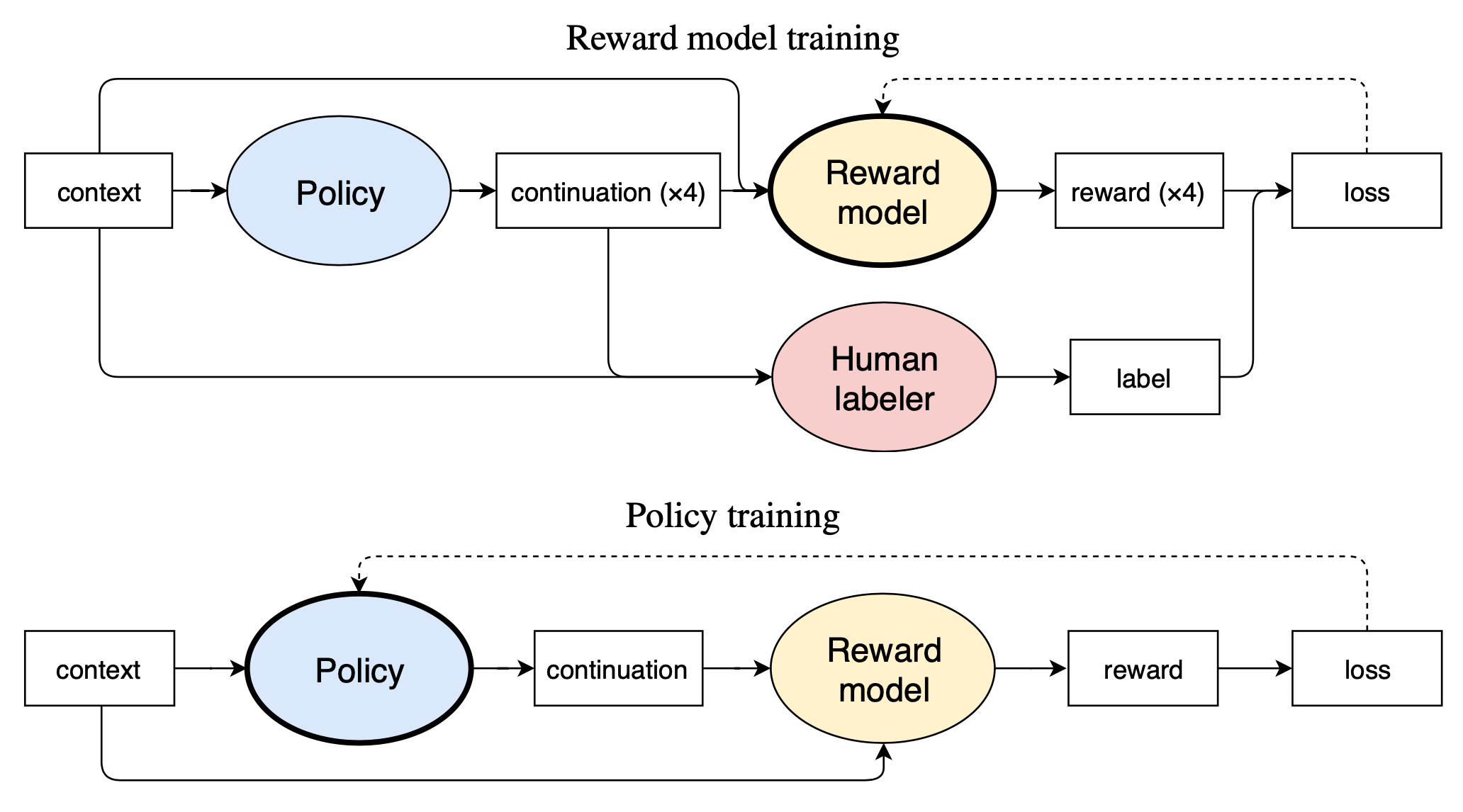Fig. 18. The overview of the training framework for fine-tuning a language model policy with reward learned from human feedback. (Image source: Ziegler et al., 2019)

The reward model is implemented by a pretrained language model with an extra random linear layer of the final embedding output. It it trained to minimize the loss:

$\mathcal{L}_\text{rm} = -\mathbb{E}_{(x, \{y_i\}, b) \sim \mathcal{D}} \Big[ \log \frac{\exp(R_\psi(x, y_b))}{\sum_i \exp(R_\psi(x, y_i))} \Big]$

To keep the scale consistent during training, the reward model is normalized to have mean 0 and variance 1.

During RL fine-tuning, the policy $$\pi$$, initialized by a pretrained language model $$p$$, is optimized via PPO with the above learned reward model. To avoid the policy’s deviating from its original behavior too much, a KL penalty is added:

$R(x, y) = R_\psi(x, y) - \beta\log\frac{\pi(y \vert x)}{p(y \vert x)}$

If running online data collection, human label collection process is continued during RL fine-tuning and thus the human labelers can review results generated by the latest policy. The number of human labels are evenly spread out during the training process. Meanwhile the reward model is also retrained periodically. Online data collection turns out to be important for the summarization task but not for the text continuation task. In their experiments, jointly training the reward model and the policy with shared parameters did not work well and can lead to overfitting due to the big imbalance between dataset sizes.

In the following work (Stiennon et al., 2020), the human label collection was further simplified to select the best option between a pair of summaries, $$y_b \in\{y_0, y_1\}$$ The reward model loss was updated to optimize the log odds of the selected summary:

$\mathcal{L}_\text{rm} = \mathbb{E}_{(x, y_0, y_1, b)\sim\mathcal{D}} [\log(\sigma(r_\theta(x, y_b) − r_\theta(x, y_{1−b})))]$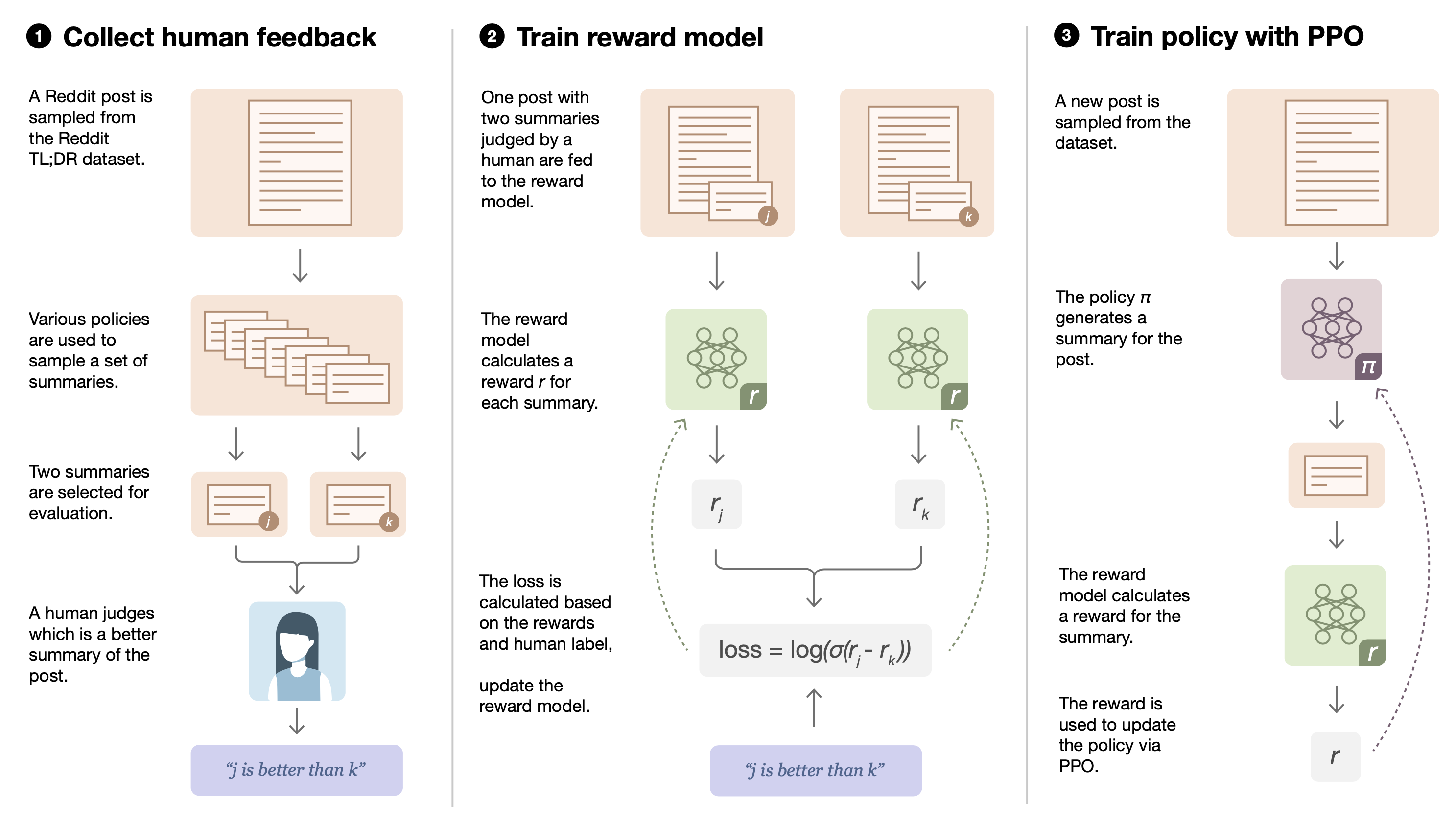Fig. 19. The overview of fine-tuning the language model policy from human feedback for summarization, including (1) human feedback collection, (2) reward model training, and (3) policy training. (Image source: Stiennon et al., 2020)

### Guided Fine-tuning with Steerable Layer

Instead of fine-tuning the entire model, only fine-tuning a small extra set of parameters while the base model stays fixed is computationally cheaper.

In computer vision, plug-and-play generative networks (PPGN; Nguyen et al., 2017) generate images with different attributes by plugging a discriminator $$p(a \vert x)$$ into a base generative model $$p(x)$$. Then the sample with a desired attribute $$a$$ can be sampled from $$p(x \vert a) \propto p(a \vert x)p(x)$$. Inspired by PPGN, the plug-and-play language model (PPLM; Dathathri et al., 2019) combines one or multiple simple attribute models with a pretrained language model for controllable text generation.

Given an attribute $$a$$ and the generated sample $$x$$, let an attribute model be $$p(a\vert x)$$. To control content generation, the current latent representation at time $$t$$, $$H_t$$ (containing a list of key-value pairs per layer), can be shifted by $$\Delta H_t$$ in the direction of the sum of two gradients:

• One toward higher log-likelihood of the attribute $$a$$ under $$p(a \vert x)$$ — so that the output content acquires a desired attribute.
• The other toward higher log-likelihood of the unmodified language model $$p(x)$$ — so that the generated text is still in fluent and smooth natural language.

To shift the output, at decoding time, PPLM runs one forward → one backward → one forward, three passes in total:

1. First a forward pass is performed to compute the likelihood of attribute $$a$$ by $$p(a\vert x)$$;
2. Let $$\Delta H_t$$ be a stepwise update to the hidden state $$H_t$$ such that $$(H_t + \Delta H_t)$$ shifts the distribution of generated text closer to having the attribute $$a$$. $$\Delta H_t$$ is initialized at zero. Then a backward pass updates the LM hidden states using normalized gradients from the attribute model $$\nabla_{\Delta H_t} \log p(a \vert H_t + \Delta H_t)$$ as $$\Delta H_t \leftarrow \Delta H_t + \alpha \frac{\nabla_{\Delta H_t} \log p(a|H_t + \Delta H_t)}{\| \nabla_{\Delta H_t} \log p(a|H_t + \Delta H_t) \|^\gamma}$$ where $$\gamma$$ is a normalization scaling coefficient, set per layer. $$\alpha$$ is step size. This update can be repeated $$m \in [3, 10]$$ times
3. The final forward pass recomputes a new distribution over the vocabulary, generated from the updated latents $$\tilde{H}_t = H_t + \Delta H_t$$. The next token is sampled from the updated distribution.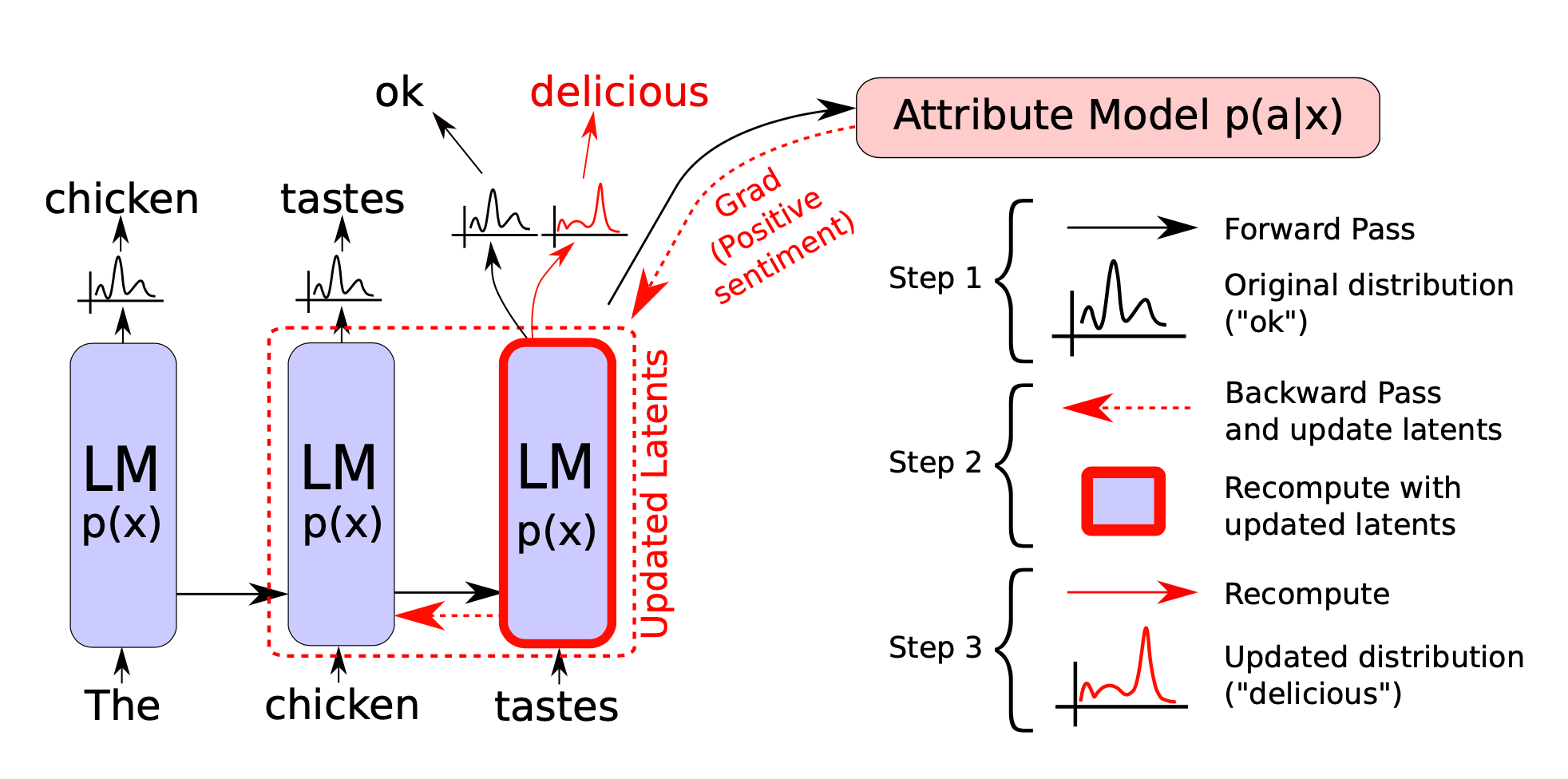Fig. 20. The overview of how PPLM runs three passes to update the model output to increase the likelihood of a desired attribute. (Image source: Dathathri et al., 2019)

Multiple attribute models can be mix-and-matched during generation with customized weights, acting as a set of “control knobs”. The PPLM paper explored two types of attribute models:

1. The simplest attribution model is based on a predefined bag of words (BoW), $$\{w_1, \dots, w_k\}$$, that specifies a topic of interest.
$$\log p(a \vert x) = \log\big( \sum_{i=1}^k p_{t+1} [w_i] \big)$$
To encourage the model to output the desired words at least once but not at every step, they normalize the gradient by the maximum gradient norm.
Interestingly, they found that increasing the probability of generating words in the bag also increases the probability of generating related but not identical words about the same topic.
2. The discriminator attribute models are based on learned classifiers which define preferences by a distribution instead of hard samples.

To ensure the fluency in language, PPLM applied two additional designs:

1. Minimizing the KL diverge between modified and unmodified LM, commonly seen in other RL fine-tuning approaches (see above).
2. It performs post-norm fusion to constantly tie the generated text to the unconditional LM $$p(x)$$, $$x_{t+1} \sim \frac{1}{\beta}(\tilde{p}_{t+1}^{\gamma_\text{gm}} p_{t+1}^{1-\gamma_\text{gm}})$$, where $$p_{t+1}$$ and $$\tilde{p}_{t+1}$$ are the unmodified and modified output distributions, respectively. $$\beta$$ is a normalizing factor. $$\gamma_\text{gm} \in [0.8, 0.95]$$ balances between prediction from before and after models.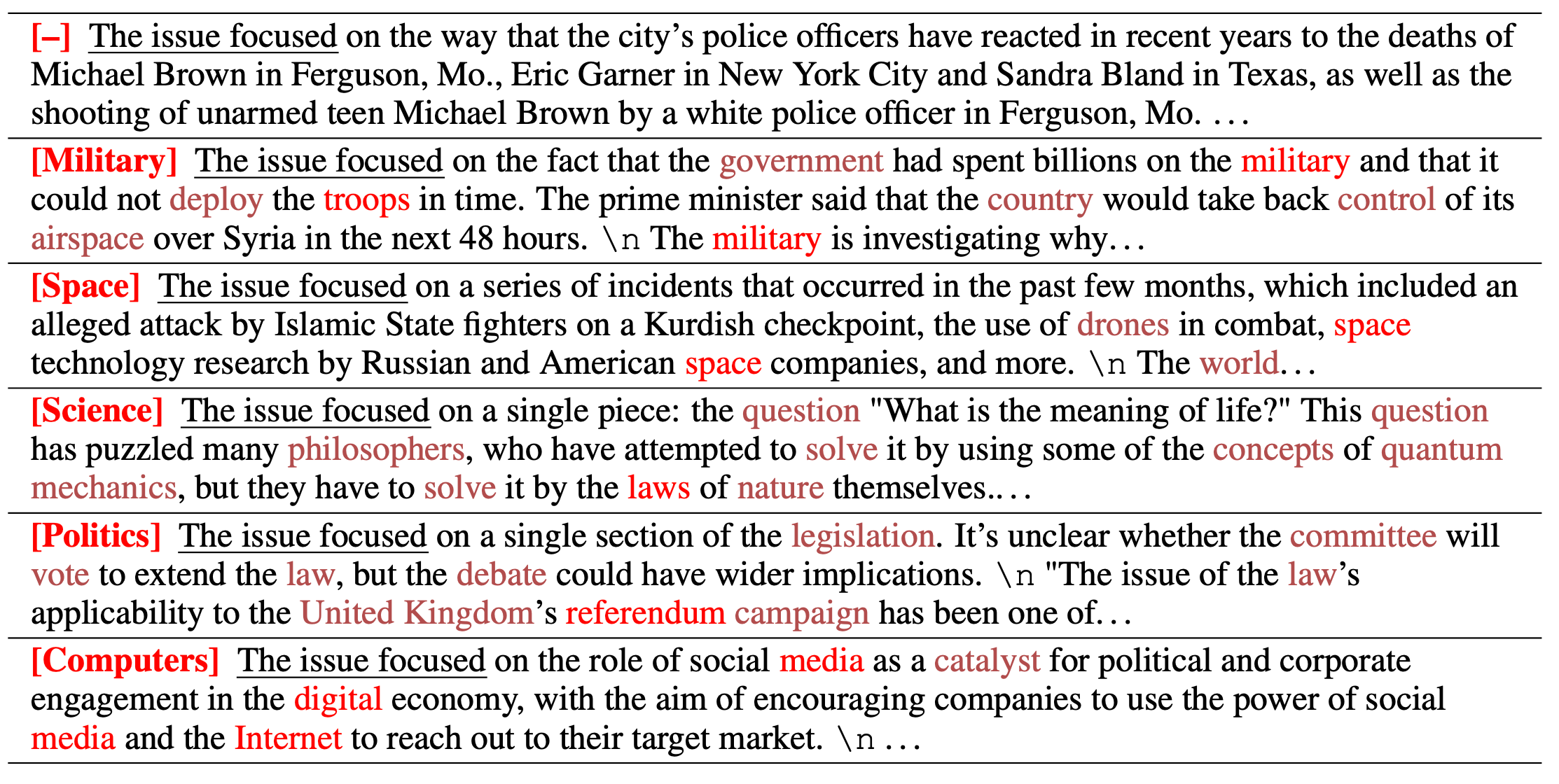Fig. 21. Examples of controllable text generation by PPLM. (Image source: Dathathri et al., 2019)

Interestingly, they found a large variance in the extent of controllability across topics. Some topics (religion, science, politics) are easier to control for compared to others (computers, space).

One obvious drawback of PPLM is that due to multiple passes at every decoding step, the test time computation becomes much more expensive.

Similar to PPLM, DELOREAN (DEcoding for nonmonotonic LOgical REAsoNing; Qin et al., 2020) incorporates the future context by back-propagation. Given input text $$\mathbf{x}$$, DELOREAN aims to generate continuation completion $$\mathbf{y} = [y_1, \dots, y_N]$$ such that $$y$$ satisfies certain constraints defined by a context $$z$$. To keep the generation differentiable, a soft representation of $$y$$ is tracked, $$\tilde{\mathbf{y}}=(\tilde{y}_1, \dots, \tilde{y}_N)$$ where $$\tilde{y}_i \in \mathbb{R}^V$$ are logits over the vocabulary. $$\tilde{\mathbf{y}}^{(t)}$$ is the soft representation at iteration $$t$$.

Given the representation $$\tilde{y}^{(t-1)}$$ at iteration $$t$$, it runs the following procedures:

1. Backward: The constraint is represented as a loss function $$\mathcal{L}(\mathbf{x}, \tilde{\mathbf{y}}^{(t-1)}, z))$$. The logits are updated via gradient descent: $$\tilde{y}^{(t), b}_n = \tilde{y}_n^{(t-1)} - \lambda \nabla_{\tilde{y}_n} \mathcal{L}(\mathbf{x}, \tilde{\mathbf{y}}^{(t-1)}, z)$$.
2. Forward: Run forward pass to ensure the generated text is fluent. $$\tilde{y}^{(t),f}_n = \text{LM}(\mathbf{x}, \tilde{\mathbf{y}}^{(t)}_{1:n-1})$$.
3. Then linearly combine two logits together to create a new representation $$\tilde{y}^{(t)}_n = \gamma \tilde{y}^{(t), f}_n + (1-\gamma) \tilde{y}^{(t), b}_n$$. Note that each $$\tilde{y}^{(t)}_n$$ is needed to sample the next $$\tilde{y}^{(t),f}_{n+1}$$.

Side-tuning (Zhang et al., 2019) trains a light-weighted side network that learns a residual on top of the original model outputs without modifying the pre-trained model weights. Unlike PPLM, no gradient update is applied on the hidden states. It is a simple yet effective approach for incremental learning. The base model is treated as a black-box model and does not necessarily have to be a neural network. Side-tuning setup assumes the base and side models are fed exactly the same input and the side model is independently learned.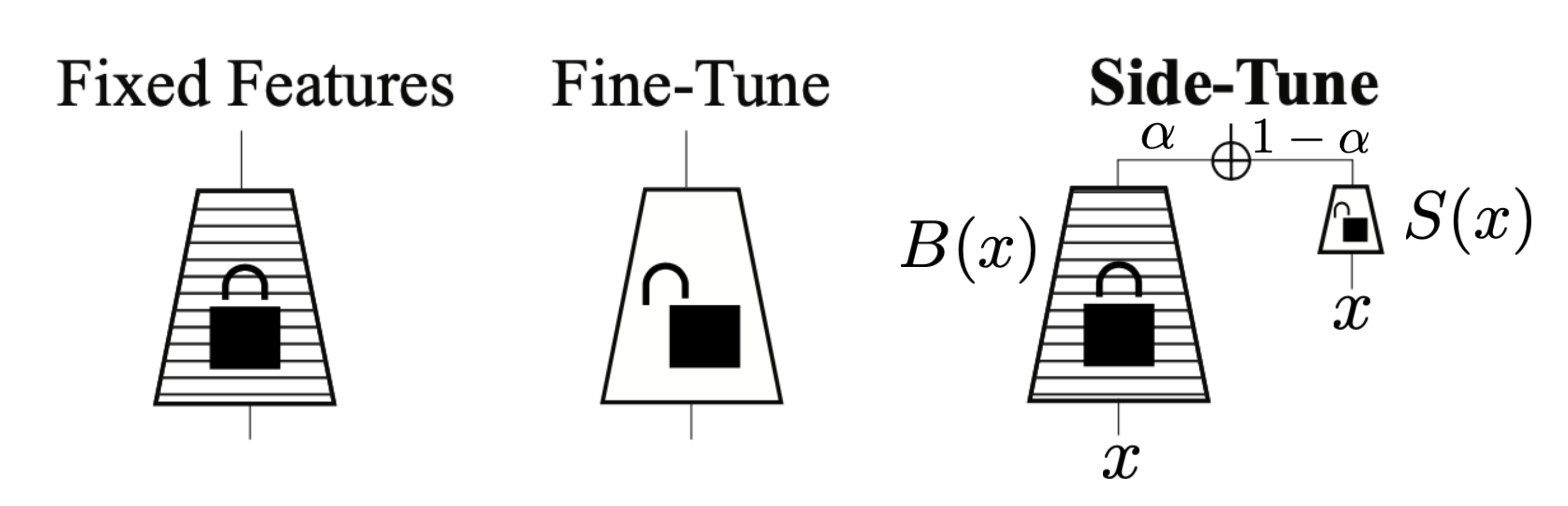Fig. 22. Comparison of fixed weights, fine-tuning and side-tuning. (Image source: Zhang et al., 2019)

The paper explored different strategies of fusing predictions from the base and side models: product is the worst while sum ($$\alpha$$-blending), MLP, and FiLM are comparable. Side-tuning is able to achieve better performance, when it is trained with intermediate amounts of data and when the base network is large.

Auxiliary tuning (Zeldes et al., 2020) supplements the original pre-trained model with an auxiliary model that shifts the output distribution according to the target task. The base and auxiliary model outputs are merged on the logits level. The combined model is trained to maximize the likelihood $$p(x_t\vert x_{<t}, z)$$ of target output.

The conditional probability of $$p(x_t\vert x_{<t}, z)$$ can be decomposed into two parts:

1. $$p(x_t\vert x_{<t})$$ assigns high probabilities to fluent sequences of tokens;
2. a shift on $$p(x_t\vert x_{<t})$$ towards $$p(x_t\vert x_{<t}, z)$$.
$p(x_t\vert x_{<t}, z) = \text{softmax}(\text{logits}_\text{LM}(x_t \vert x_{<t}) + \text{logits}_\text{aux}(x_t \vert x_{<t}, z))$

By Bayesian rule, we have

$p(x_t\vert x_{<t}, z) = \frac{p(z \vert x_{\leq t})}{p(z)} p(x_t \vert x_{<t}) \propto p(z \vert x_{\leq t}) p(x_t \vert x_{<t})$

And therefore the auxiliary model $$\text{logits}_\text{aux}(x_t \vert x_{<t}, z))$$ effectively should learn to predict $$p(z \vert x_{\leq t})$$. In the experiments of Zeldes et al., 2020, the auxiliary model can re-use the intermediate layers of the pre-trained LM for feature extraction.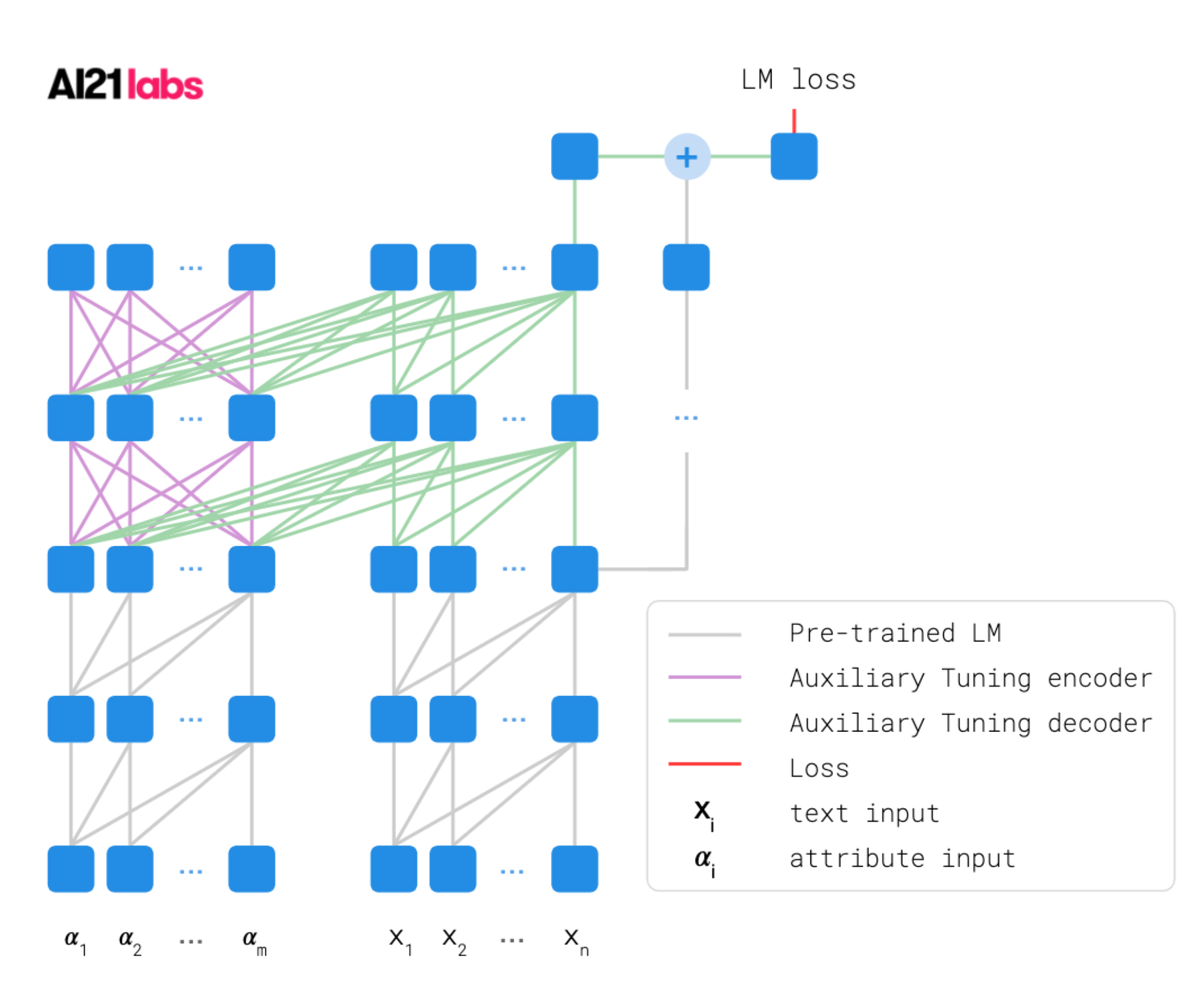Fig. 23. The auxiliary model is trained by reusing features extracted from multiple layers of the base model. (Image source: Zeldes et al., 2020)

GeDi (Kruse et al., 2020) guides the text generation by Generative Discriminator. The discriminator is implemented as a class conditional language model (CC-LM), $$p_\theta(x_{1:t} \vert z)$$. The discriminator guides generation at each decoding step by computing classification probabilities for all possible next tokens via Bayes rule by normalizing over two contrastive class-conditional distributions:

1. One conditioned on the control code $$z$$ for desired attribute.
2. The other conditioned on the anti-control code $$\bar{z}$$ for undesired attributes.

GeDi relies on the contract between $$p_\theta(x_{1:t} \vert z)$$ and $$p_\theta(x_{1:t} \vert \bar{z})$$ to compute the probability of the sequence belonging to the desired class. The discriminator loss is to maximize the probability of desired attribute $$z$$:

\begin{aligned} p_\theta(z \vert x_{1:t}) &= \frac{p(z) p_\theta(x_{1:\tau} \vert z)^{\alpha/\tau}}{\sum_{z' \in \{z, \bar{z}\}} p(z') p_\theta(x_{1:\tau} \vert z')^{\alpha/\tau} } \\ \mathcal{L}_\text{desc} &= -\frac{1}{N} \sum_{i=1}^N \log p_\theta(z^{(i)} \vert x^{(i)}_{1:\tau_i}) \\ &= -\frac{1}{N} \sum_{i=1}^N \log \frac{p(z) p_\theta(x^{(i)}_{1:\tau_i} \vert z^{(i)})^{\alpha/t_i}}{\sum_{z' \in \{z, \bar{z}\} } p(z')p_\theta(x^{(i)}_{1:\tau_i} \vert z')^{\alpha/\tau_i}} \end{aligned}

where $$p(z) = \exp(b_z) / \sum_{z'} \exp(b_{z'})$$ and $$b_z$$ is a learned class prior. The probabilities are normalized by the current sequence length $$\tau$$ to robustify generation sequences of variable lengths. $$\tau_i$$ is the sequence length of the $$i$$-th input $$x^{(i)}$$ in the dataset.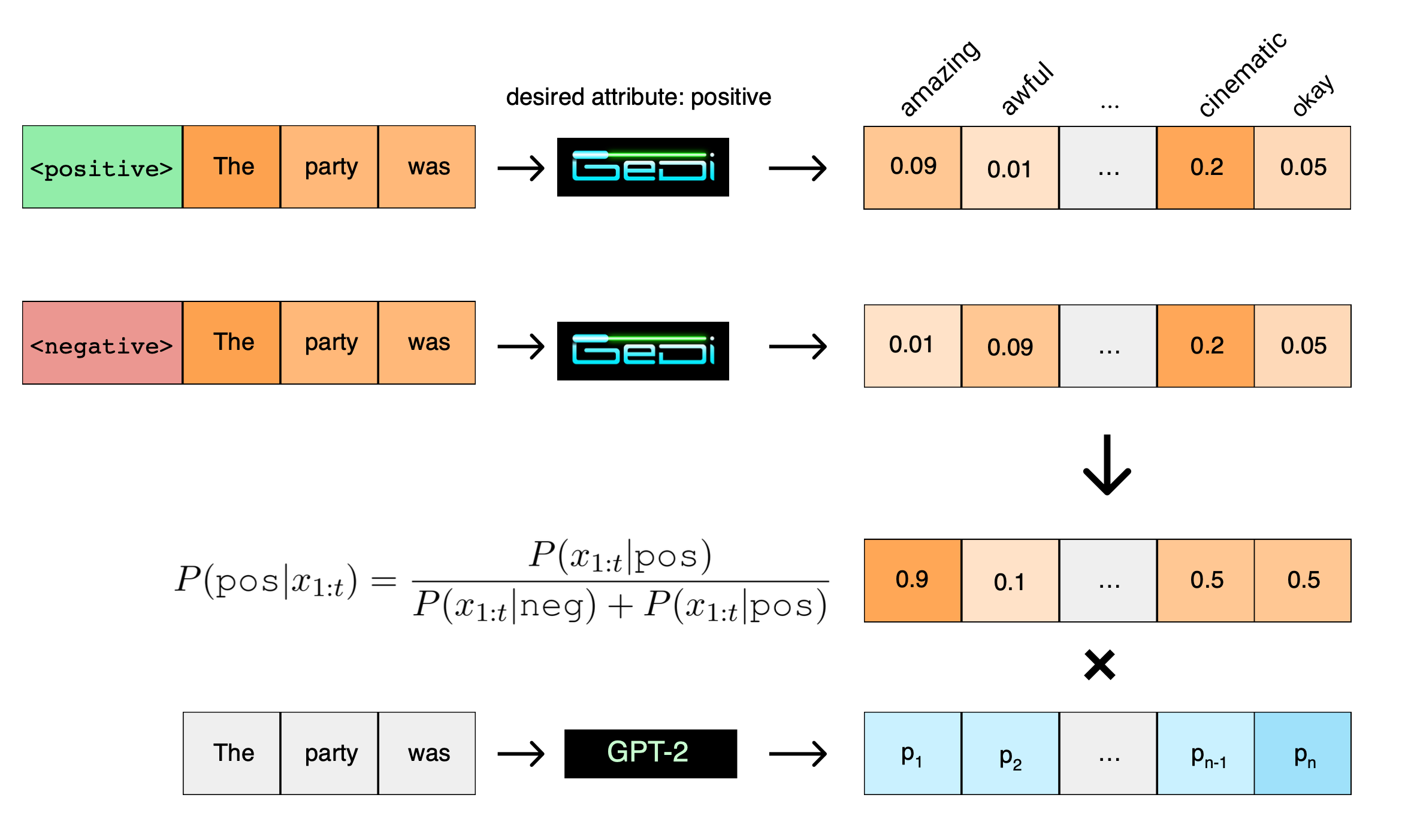Fig. 24. An illustration of how GeDi works via Bayesian rule. (Image source: Kruse et al., 2020)

They finetuned a GPT2-medium model with control code similar to how CTRL is trained to form a CC-LM using a linear combination of discriminative loss and generative loss. This discriminator model is then used as GiDe to guide generation by a larger language model like GPT2-XL.

One way of decoding from GeDi is to sample from a weighted posterior $$p^w(x_{t+1}\vert x_{1:t}, z) \propto p(z \vert x_{1:t+1})^w p(x_{t+1} \vert x_{1:t})$$ where $$w>1$$ applies additional bias toward the desired class $$z$$. In the sampling process, only tokens with the class or next-token probability larger than a certain threshold are selected.

GeDi guided generation in their experiments showed strong controllability and ran 30x faster than PPLM.

### Distributional Approach

Generation with Distributional Control (GDC; Khalifa, et al. 2020) frames controlled text generation as the optimization of a probability distribution with a constraint. It involves two major steps.

Step 1: Learn a EBM of the target model

Let’s label a pretrained LM as $$a$$ and a target LM with desired features as $$p$$. The desired features can be defined by a set of pre-defined real-valued feature functions $$\phi_i(x), i=1,\dots,k$$ over $$x \in X$$, denoted as a vector $$\boldsymbol{\phi}$$. When sequences $$x \in X$$ are sampled according to the desired model $$p$$, the expectations of features $$\mathbb{E}_{x\sim p}\boldsymbol{\phi}(x)$$ should be close to $$\bar{\boldsymbol{\mu}}$$ , named “moment constraints”. The feature function $$\phi_i$$ can have distinct values (e.g. identity function for binary classifier) or continuous probabilities. In the meantime, the fine-tuned model $$p$$ should not diverge from $$a$$ too much by maintaining a small KL divergence measure.

In summary, given a pretrained model $$a$$, we would like to find a target model $$p$$ such that:

\begin{aligned} \bar{\boldsymbol{\mu}} &= \mathbb{E}_{x\sim p}\boldsymbol{\phi}(x) \\ p &= \arg\min_{c \in \mathcal{C}} D_\text{KL}(c, a) \end{aligned}

where $$\mathcal{C}$$ is the set of all distributions over $$X$$ that satisfy the moment constraints.

According to theorems in Information Geometry, $$p$$ can be approximated by an EBM (energy-based model; an unnormalized probability distribution) $$P$$ in the form of exponential function, such that $$p(x) \propto P(x)$$ and $$p(x)=\frac{1}{Z}P(x)$$ where $$Z=\sum_x P(x)$$. The energy-based model can be approximated by: $$P(x)=a(x)\exp\big(\sum_i \lambda_i \phi_i(x)\big)=a(x)\exp(\boldsymbol{\lambda}\cdot\boldsymbol{\phi}(x))$$ Let’s define importance weight $$w(x, \boldsymbol{\lambda}) = \frac{P(x)}{a(x)} = \exp\langle\boldsymbol{\lambda}\cdot\boldsymbol{\phi}(x)\rangle$$. Given a large number of sequences sampled from the pretrained model $$x_1, \dots, x_N \sim a(x)$$,

\begin{aligned} \mu(\boldsymbol{\lambda}) &= \mathbb{E}_{x\sim p}\boldsymbol{\phi}(x) = \mathbb{E}_{x\sim a} \frac{p(x)}{a(x)}\boldsymbol{\phi}(x) = \frac{1}{Z}\mathbb{E}_{x\sim a} w(x, \boldsymbol{\lambda}) \boldsymbol{\phi}(x) \\ &= \frac{\mathbb{E}_{x\sim a} w(x, \boldsymbol{\lambda}) \boldsymbol{\phi}(x)}{\sum_{x\in X} P(x)} = \frac{\mathbb{E}_{x\sim a} w(x, \boldsymbol{\lambda}) \boldsymbol{\phi}(x)}{\sum_{x\in X} w(x, \boldsymbol{\lambda})a(x)} = \frac{\mathbb{E}_{x\sim a} w(x, \boldsymbol{\lambda}) \boldsymbol{\phi}(x)}{\mathbb{E}_{x\sim a} w(x, \boldsymbol{\lambda})} \\ &\simeq \frac{\sum_{i=1}^N w(x_i,\boldsymbol{\lambda}) \boldsymbol{\phi}(x_i)}{\sum_{i=1}^N w(x_i, \boldsymbol{\lambda})} = \frac{\sum_{i=1}^N \exp\langle\boldsymbol{\lambda}\cdot\boldsymbol{\phi}(x)\rangle \boldsymbol{\phi}(x_i)}{\sum_{i=1}^N \exp\langle\boldsymbol{\lambda}\cdot\boldsymbol{\phi}(x)\rangle} \end{aligned}

Using SGD over the objective $$\|\boldsymbol{\mu}(\boldsymbol{\lambda}) - \bar{\boldsymbol{\mu}}\|^2_2$$, we can obtain an estimated value for $$\boldsymbol{\lambda}$$ and a representation of $$P(x)=a(x)\exp\langle\boldsymbol{\lambda}\cdot\boldsymbol{\phi}(x)\rangle$$. $$P(x)$$ is a sequential EBM because $$a$$ is an autoregressive model.

Step 2: Learn the target probability distribution

The EBM $$P(x)$$ can compute ratios of probabilities of two sequences, but cannot sample from $$p(x)$$ with knowing $$Z$$. In order to sample from a sequential EBM, the paper proposed to use Distributional Policy Gradient (DPG; but not this DPG) with the objective to obtain an autoregressive policy $$\pi_\theta$$ to approximate a target distribution $$p$$ by minimizing the cross entropy $$H(p, \pi_\theta)$$. DPG runs through a sequence of iterations. Within each iteration, the proposed distribution $$q$$ is used for sampling and we can correct the cross entropy loss with importance weights too:

\begin{aligned} \nabla_\theta H(p, \pi_\theta) &= - \nabla_\theta \mathbb{E}_{x\sim p} \log \pi_\theta(x) = - \mathbb{E}_{x\sim p} \nabla_\theta \log \pi_\theta(x) \\ &= - \mathbb{E}_{x\sim q} \frac{p(x)}{q(x)} \nabla_\theta \log \pi_\theta(x) = - \frac{1}{Z}\mathbb{E}_{x\sim q} \frac{P(x)}{q(x)} \nabla_\theta \log \pi_\theta(x) \end{aligned}

To learn such a $$\pi_\theta$$, the paper adopts a KL-adaptive version of DPG: It only updates $$q$$ when the estimated policy $$\pi_\theta$$ gets closer to $$p$$. This adaptive step is important for fast convergence.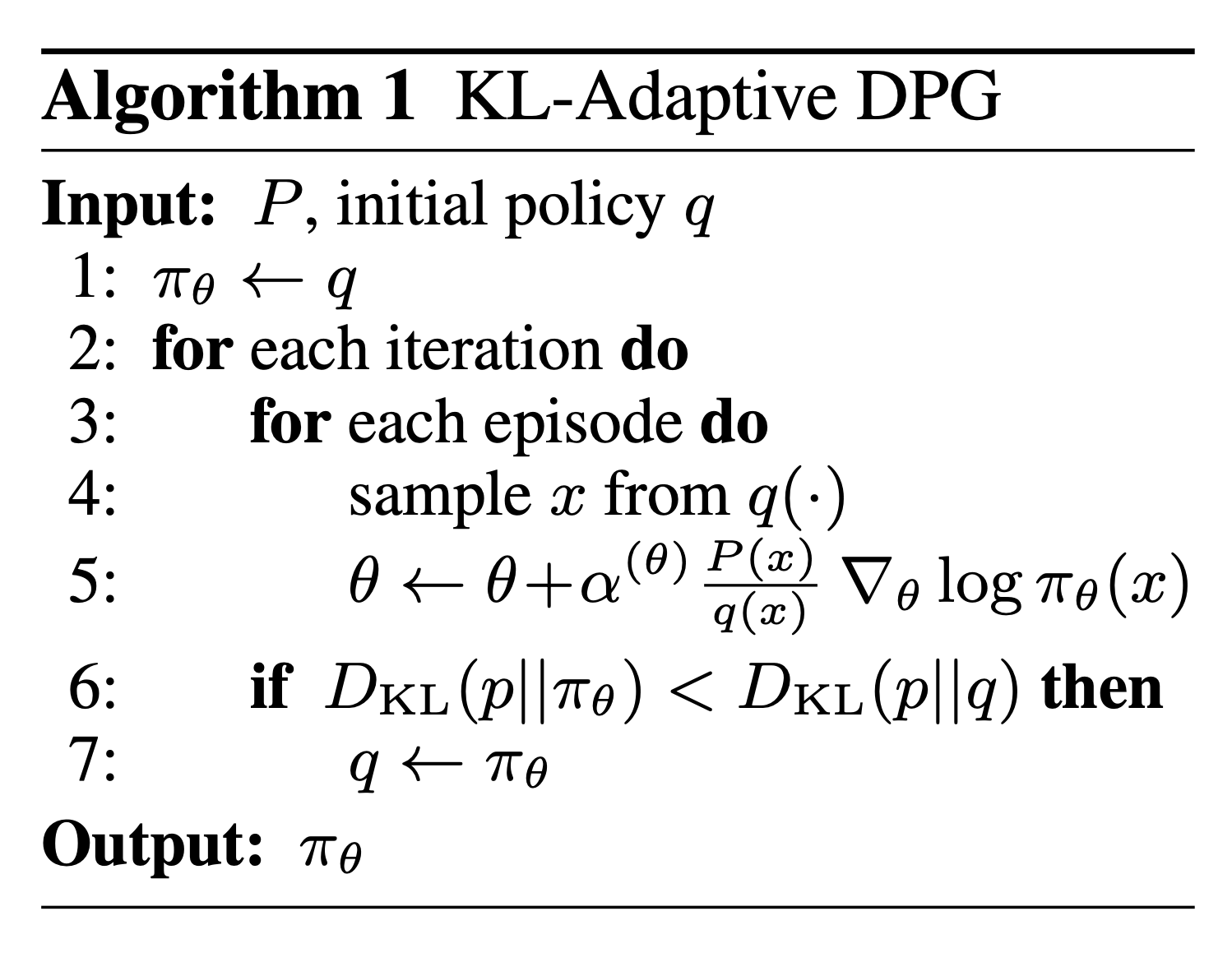Fig. 25. The algorithm of distributional policy gradient to make it possible to sample from a EBM $$P(x)$$, where $$q$$ is initialized to be $$a$$. (Image source: Khalifa, et al. 2020)

This approach can be used to model various constraints in controllable text generation:

1. Pointwise constraints: $$\phi_i$$ is a binary feature; such as constraining the presence or absence of words, or classifier-based constraints.
2. Distributional constraints: $$\phi_i$$ represents a probability distribution; such as constraining the probability of gender, topic, etc. Their experiments showed great progress in debiasing a GPT-2 model that was trained on Wikipedia Biographies corpus. The percentage of generated biographies on females increased from 7.4% to 35.6%.
3. Hybrid constraints: combine multiple constraints by simply summing them up.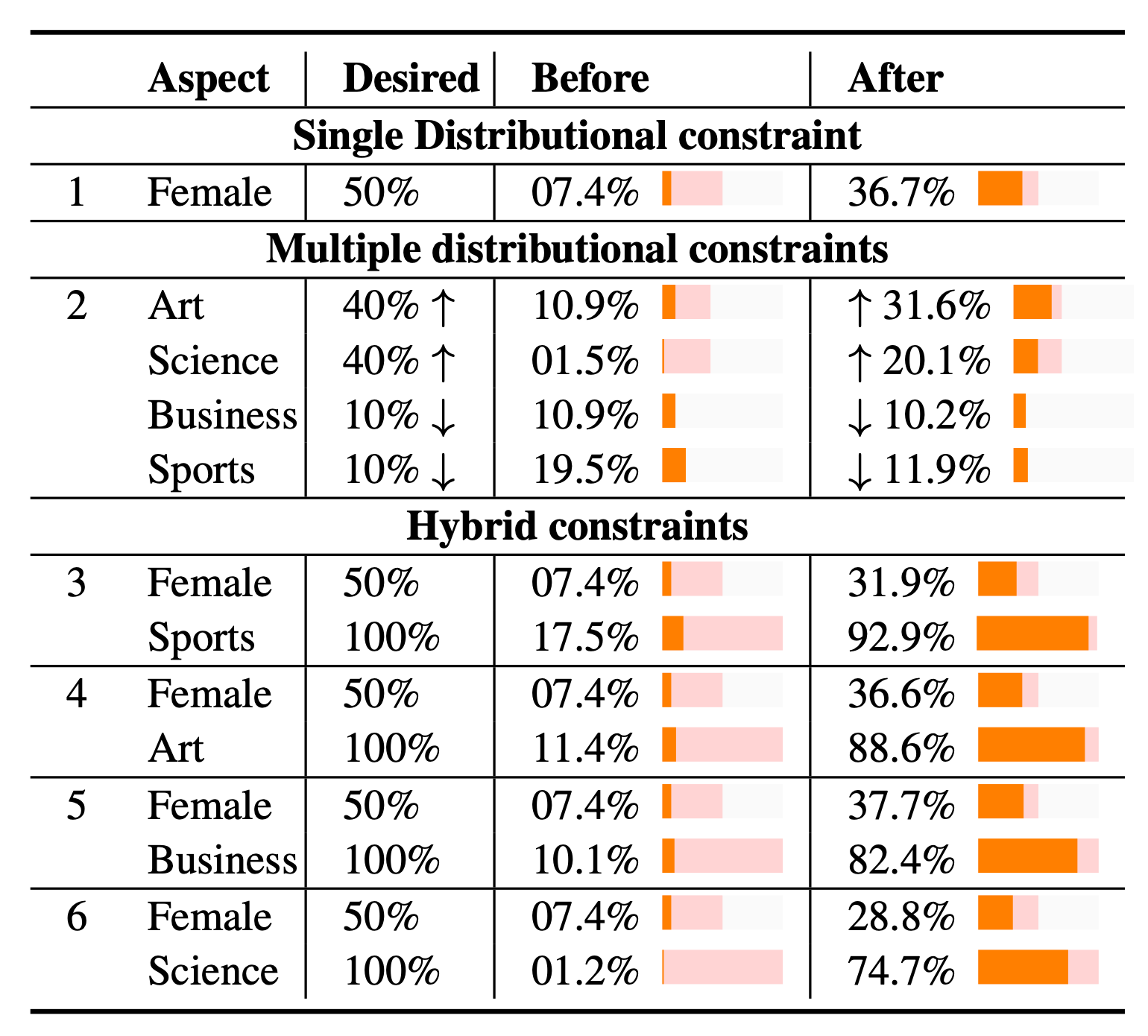Fig. 26. Debiasing experiments using GDC with various constraints. (Image source: Khalifa, et al. 2020)

Compared to other baselines, GDC using pointwise constraints diverges less from the base model $$a$$ and produces smoother curves.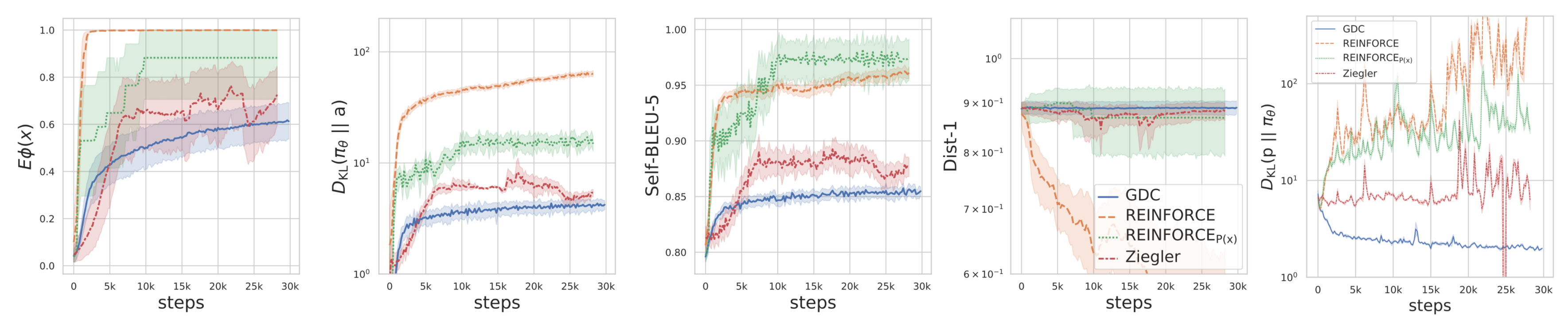Fig. 27. Compare pointwise constrained GDC with several baselines. Low Self-BLEU-5 and high Dist-1 indicate high diversity. (Image source: Khalifa, et al. 2020)

• REINFORCE that optimizes the reward $$\phi$$ directly ($$\text{REINFORCE}$$ in Fig. X.) without constraints converges fast but has a high deviation from the original model.
• REINFORCE that optimizes $$P(x)$$ ($$\text{REINFORCE}_{P(x)}$$ in Fig. X.) has low sample diversity.
• Compared to Ziegler et al., 2019 GDC has smoother learning curves and produces a richer vocabulary.

### Unlikelihood Training

The standard way of maximizing the log-likelihood loss in language model training leads to incorrect token distribution, which cannot be fixed with only smart decoding methods. Such models tend to output high-frequency words too often and low-frequency words too rarely, especially when using deterministic decoding (e.g. greedy, beam search). In other words, they are overconfident in their predictions.

Unlikelihood training (Welleck & Kulikov et al. 2019] tries to combat this and incorporates preference to unwanted content into the training objective directly. It combines two updates:

• A routine maximized likelihood update to assign true tokens with high probability;
• A new type of unlikelihood update to avoid unwanted tokens with high probability.

Given a sequence of tokens $$(x_1, \dots, x_T)$$ and a set of negative candidate tokens $$\mathcal{C}^t = \{c_1, \dots , c_m\}$$ at step $$t$$, where each token $$x_i, c_j \in \mathcal{V}$$, the combined loss for step $$t$$ is defined as:

$\mathcal{L}^t_\text{UL}(p_\theta (. \vert x_{<t}), \mathcal{C}^t) = - \alpha \cdot \underbrace{\sum_{c \in \mathcal{C}^t} \log(1 - p_\theta(c \vert x_{<t}))}_\text{unlikelihood} - \underbrace{\log p_\theta (x_t \vert x_{<t})}_\text{likelihood}$

One approach for constructing $$\mathcal{C}^t$$ is to randomly select candidates from model-generated sequences.

The unlikelihood training can be extended to be on the sequence-level, where the negative continuation is defined by a sequence of per-step negative candidate sets. They should be designed to penalize properties that we don’t like. For example, we can penalize repeating n-grams as follows:

$\mathcal{C}^t_\text{repeat-n} = \{x_t\} \text{ if }(x_{t-i}, \dots, x_{t+j}) \in x_{<t-i} \text{ for any } (j-i)=n, i\leq n \leq j.$

Their experiments used unlikelihood training to avoid repetitions in language model outputs and indeed showed better results on less repetition and more unique tokens compared to standard MLE training.

Cited as:

@article{weng2021conditional,
title   = "Controllable Neural Text Generation.",
author  = "Weng, Lilian",
journal = "lilianweng.github.io/lil-log",
year    = "2021",
url     = "https://lilianweng.github.io/lil-log/2021/01/02/controllable-neural-text-generation.html"
}


 Patrick von Platen. “How to generate text: using different decoding methods for language generation with Transformers” Hugging face blog, March 18, 2020.

 Angela Fan, et al. “Hierarchical Neural Story Generation/” arXiv preprint arXiv:1805.04833 (2018).

 Ari Holtzman et al. “The Curious Case of Neural Text Degeneration.” ICLR 2020.

 Marjan Ghazvininejad et al. “Hafez: an interactive poetry generation system.” ACL 2017.

 Ari Holtzman et al. “Learning to write with cooperative discriminators.” ACL 2018.

 Ashutosh Baheti et al. “Generating More Interesting Responses in Neural Conversation Models with Distributional Constraints.” EMNLP 2018.

 Jiatao Gu et al. “Trainable greedy decoding for neural machine translation.” EMNLP 2017.

 Kyunghyun Cho. “Noisy Parallel Approximate Decoding for Conditional Recurrent Language Model.” arXiv preprint arXiv:1605.03835. (2016).

 Marco Tulio Ribeiro et al. “Semantically equivalent adversarial rules for debugging NLP models.” ACL 2018.

 Eric Wallace et al. “Universal Adversarial Triggers for Attacking and Analyzing NLP.” EMNLP 2019. [code]

 Taylor Shin et al. “AutoPrompt: Eliciting Knowledge from Language Models with Automatically Generated Prompts.” EMNLP 2020. [code]

 Zhengbao Jiang et al. “How Can We Know What Language Models Know?” TACL 2020.

 Nanyun Peng et al. “Towards Controllable Story Generation.” NAACL 2018.

 Nitish Shirish Keskar, et al. “CTRL: A Conditional Transformer Language Model for Controllable Generation” arXiv preprint arXiv:1909.05858 (2019).[code]

 Marc’Aurelio Ranzato et al. “Sequence Level Training with Recurrent Neural Networks.” ICLR 2016.

 Yonghui Wu et al. “Google’s Neural Machine Translation System: Bridging the Gap between Human and Machine Translation.” CoRR 2016.

 Romain Paulus et al. “A Deep Reinforced Model for Abstractive Summarization.” ICLR 2018.

 Paul Christiano et al. “Deep Reinforcement Learning from Human Preferences.” NIPS 2017.

 Sanghyun Yi et al. “Towards coherent and engaging spoken dialog response generation using automatic conversation evaluators.” INLG 2019.

 Florian Böhm et al. “Better rewards yield better summaries: Learning to summarise without references.” EMNLP 2019. [code]

 Daniel M Ziegler et al. “Fine-tuning language models from human preferences.” arXiv preprint arXiv:1909.08593 (2019). [code]

 Nisan Stiennon, et al. “Learning to summarize from human feedback.” arXiv preprint arXiv:2009.01325 (2020).

 Sumanth Dathathri et al. “Plug and play language models: a simple approach to controlled text generation.” ICLR 2020. [code]

 Jeffrey O Zhang et al. “Side-tuning: Network adaptation via additive side networks” ECCV 2020.

 Ben Kruse et al. “GeDi: Generative Discriminator Guided Sequence Generation.” arXiv preprint arXiv:2009.06367.

 Yoel Zeldes et al. “Technical Report: Auxiliary Tuning and its Application to Conditional Text Generatio.” arXiv preprint arXiv:2006.16823.

 Thomas Scialom, et al. “Discriminative Adversarial Search for Abstractive Summarization” ICML 2020.

 Clara Meister, et al. “If beam search is the answer, what was the question?” EMNLP 2020.

 Xiang Lisa Li and Percy Liang. “Prefix-Tuning: Optimizing Continuous Prompts for Generation.” arXiv preprint arXiv:2101.00190 (2021).

 Lianhui Qin, et al. “Back to the Future: Unsupervised Backprop-based Decoding for Counterfactual and Abductive Commonsense Reasoning.” arXiv preprint arXiv:2010.05906 (2020).

 Muhammad Khalifa, et al. “A Distributional Approach to Controlled Text Generation” Accepted by ICLR 2021.

 Aditya Grover, et al. “Bias correction of learned generative models using likelihood-free importance weighting.” NeuriPS 2019.

 Yuntian Deng et al. “Residual Energy-Based Models for Text Generation.” ICLR 2020.

 Brian Lester et al. “The Power of Scale for Parameter-Efficient Prompt Tuning.” arXiv preprint arXiv:2104.08691 (2021).

 Xiao Liu et al. “GPT Understands, Too.” arXiv preprint arXiv:2103.10385 (2021).

 Welleck & Kulikov et al. “Neural Text Generation with Unlikelihood Training” arXiv:1908.04319 (2019).